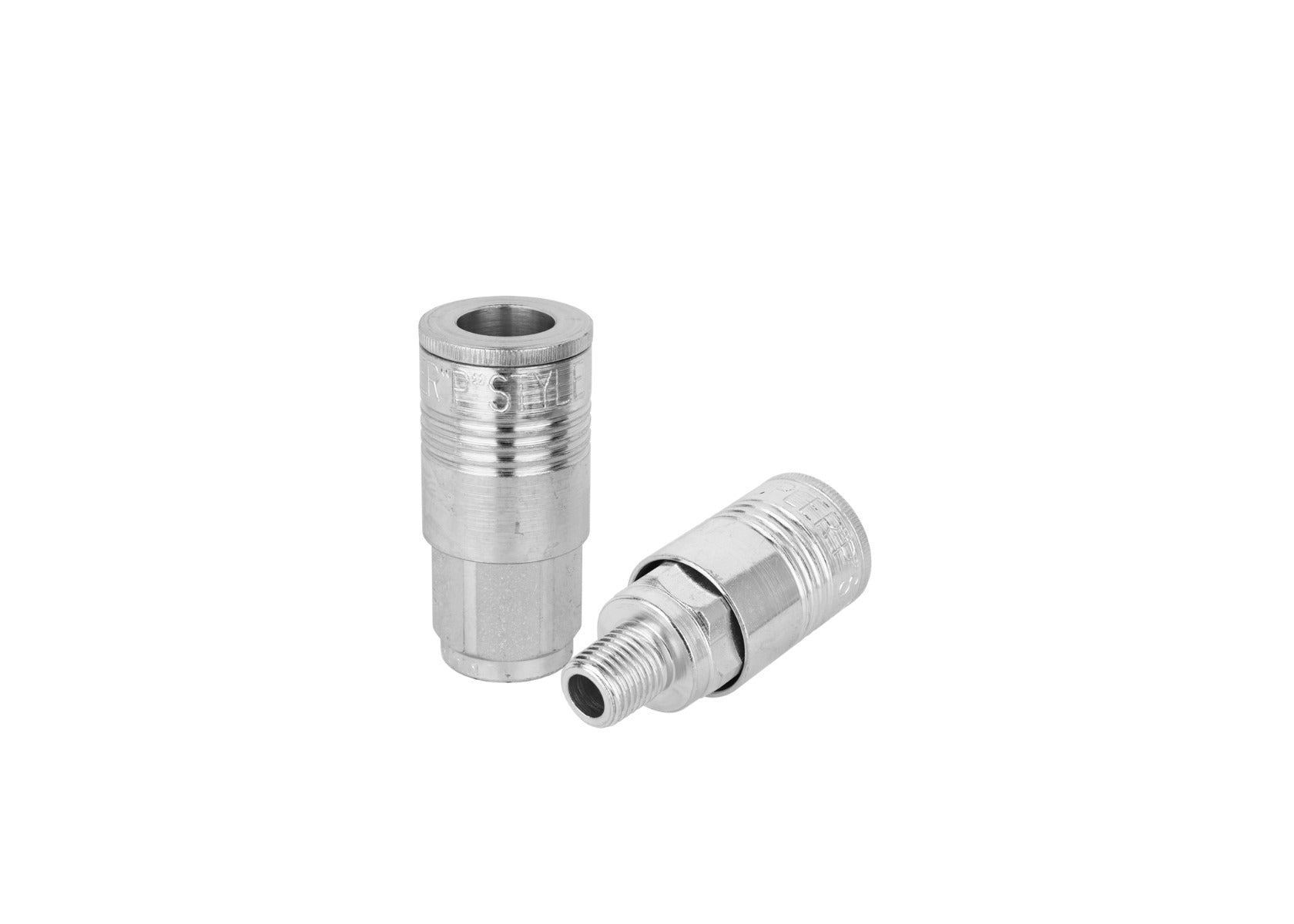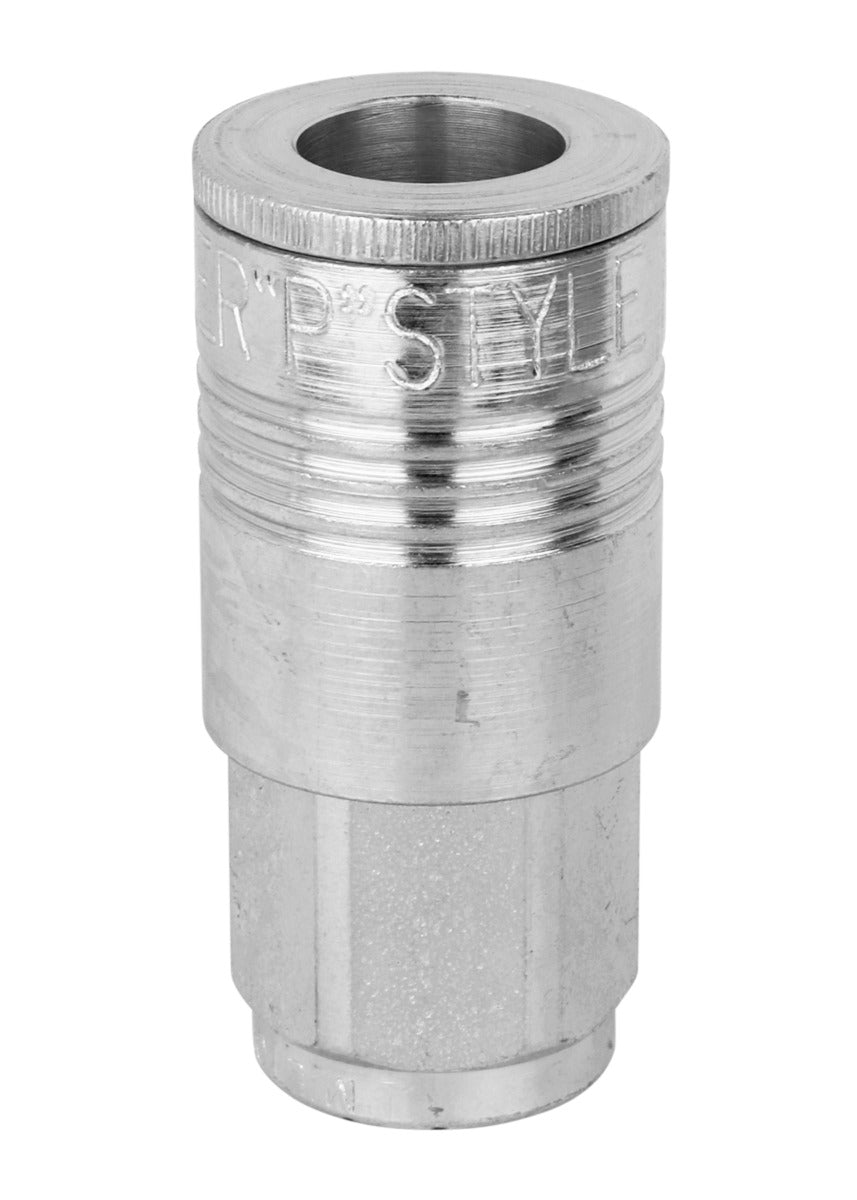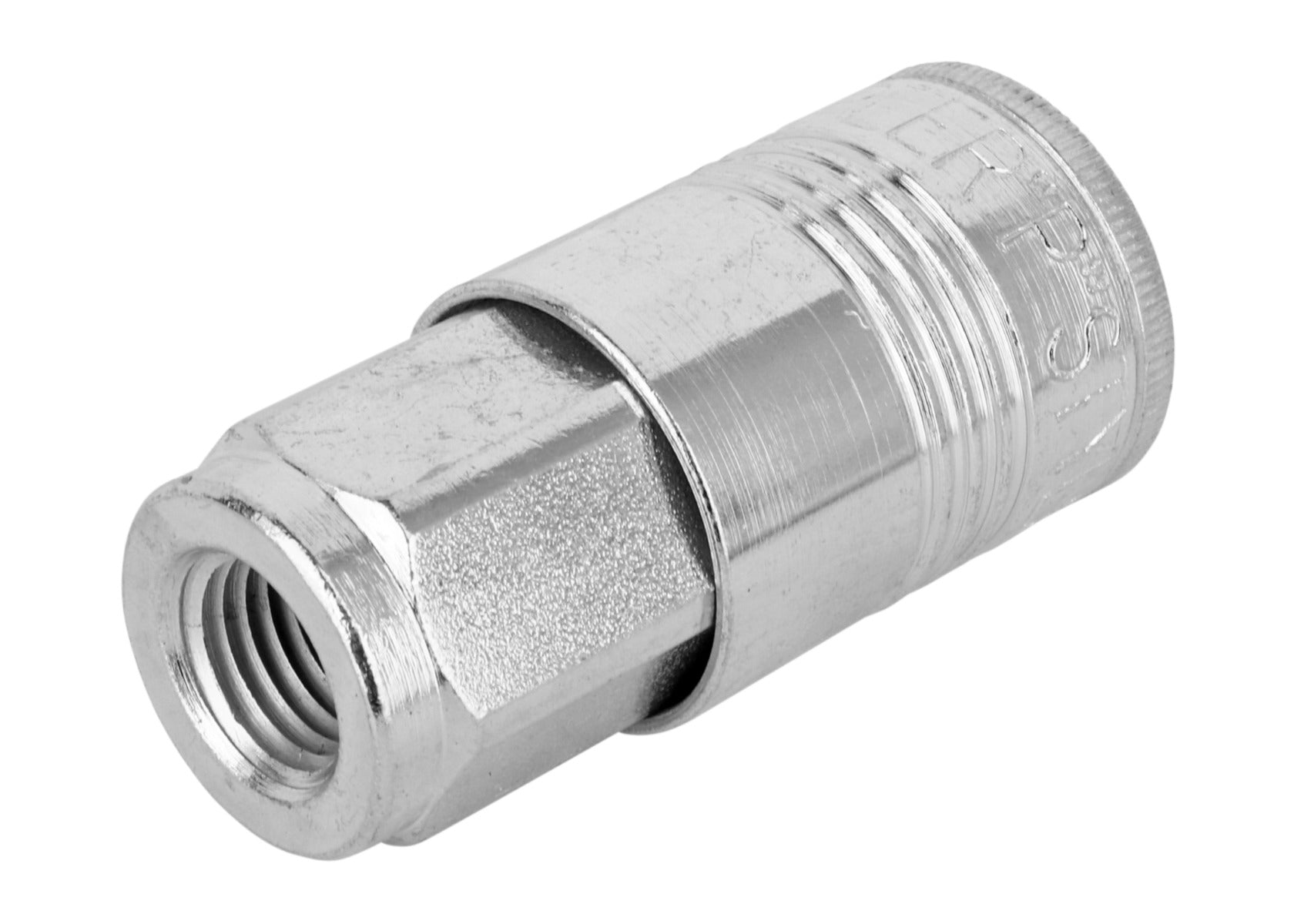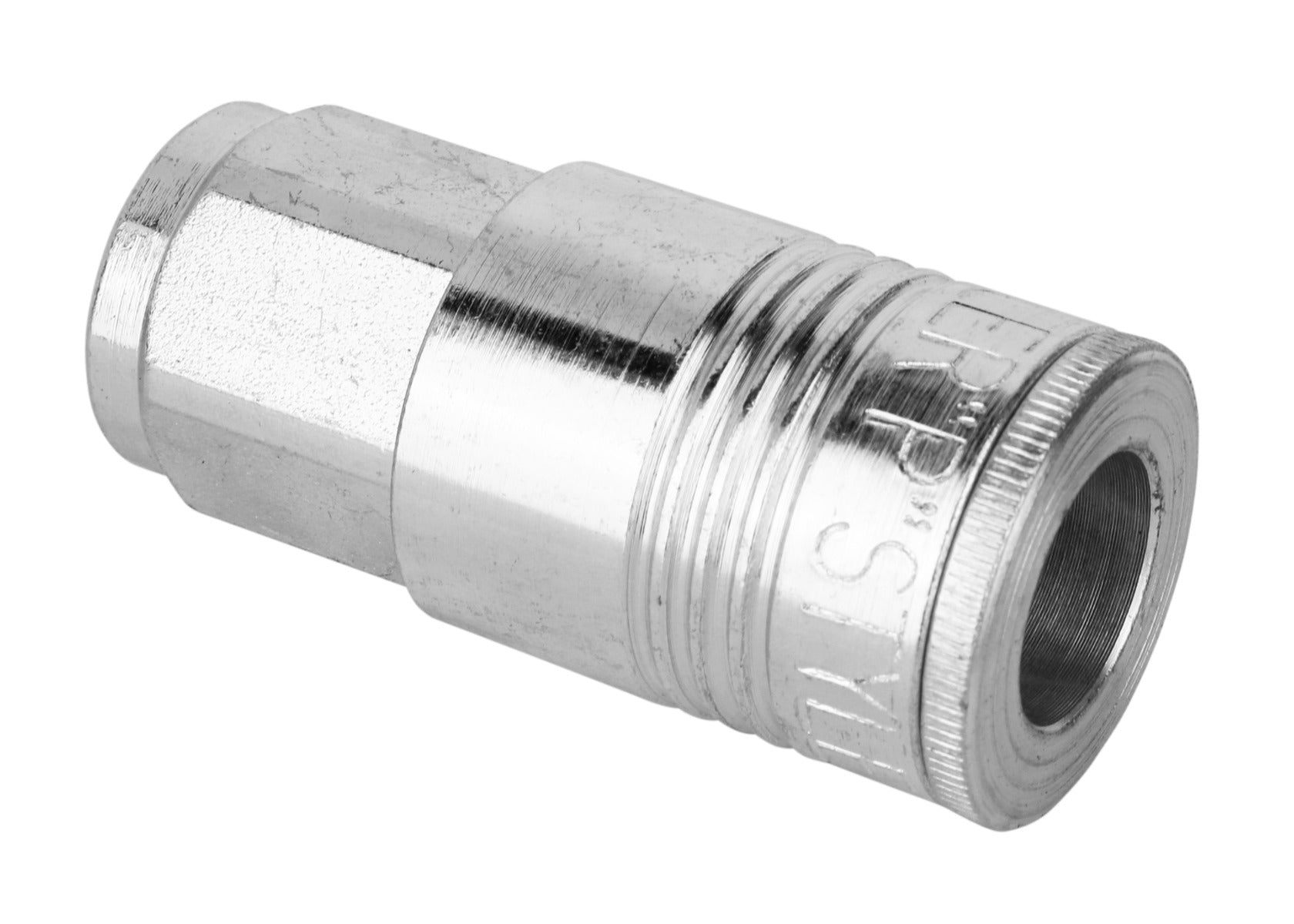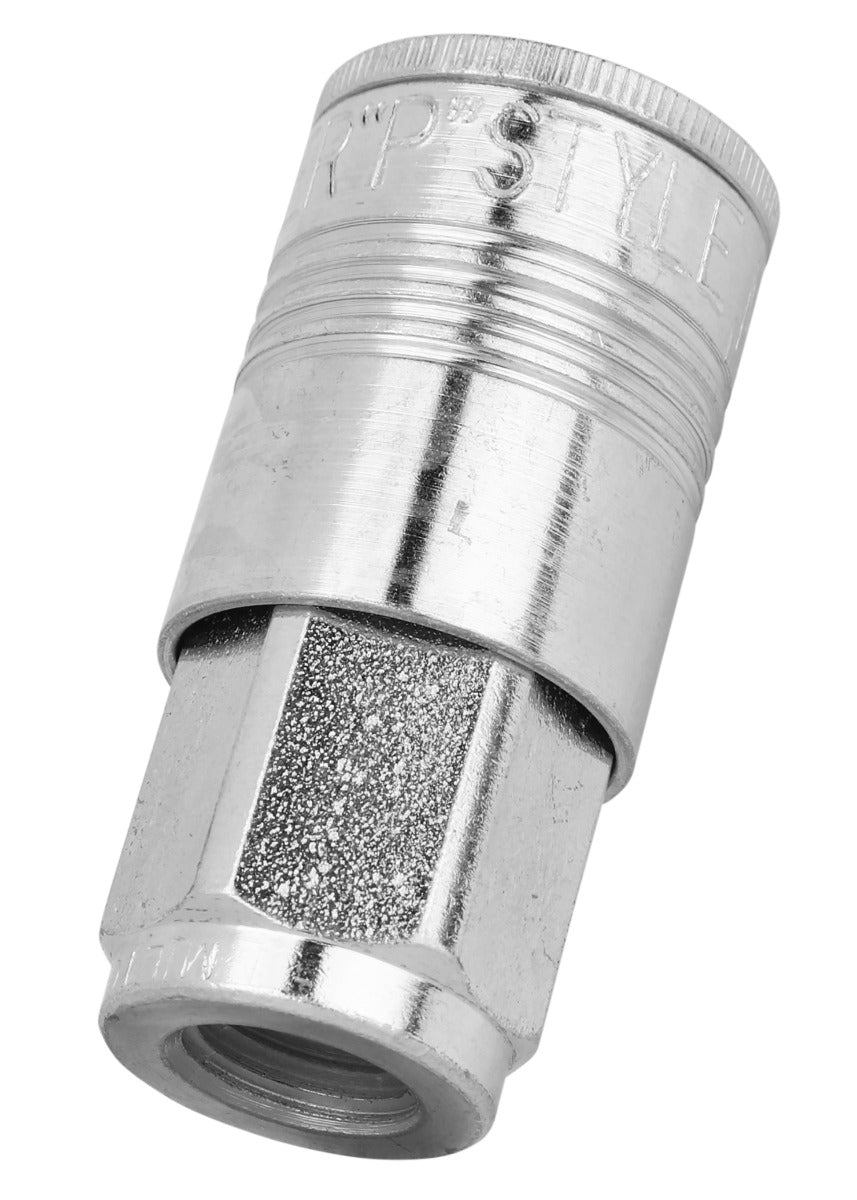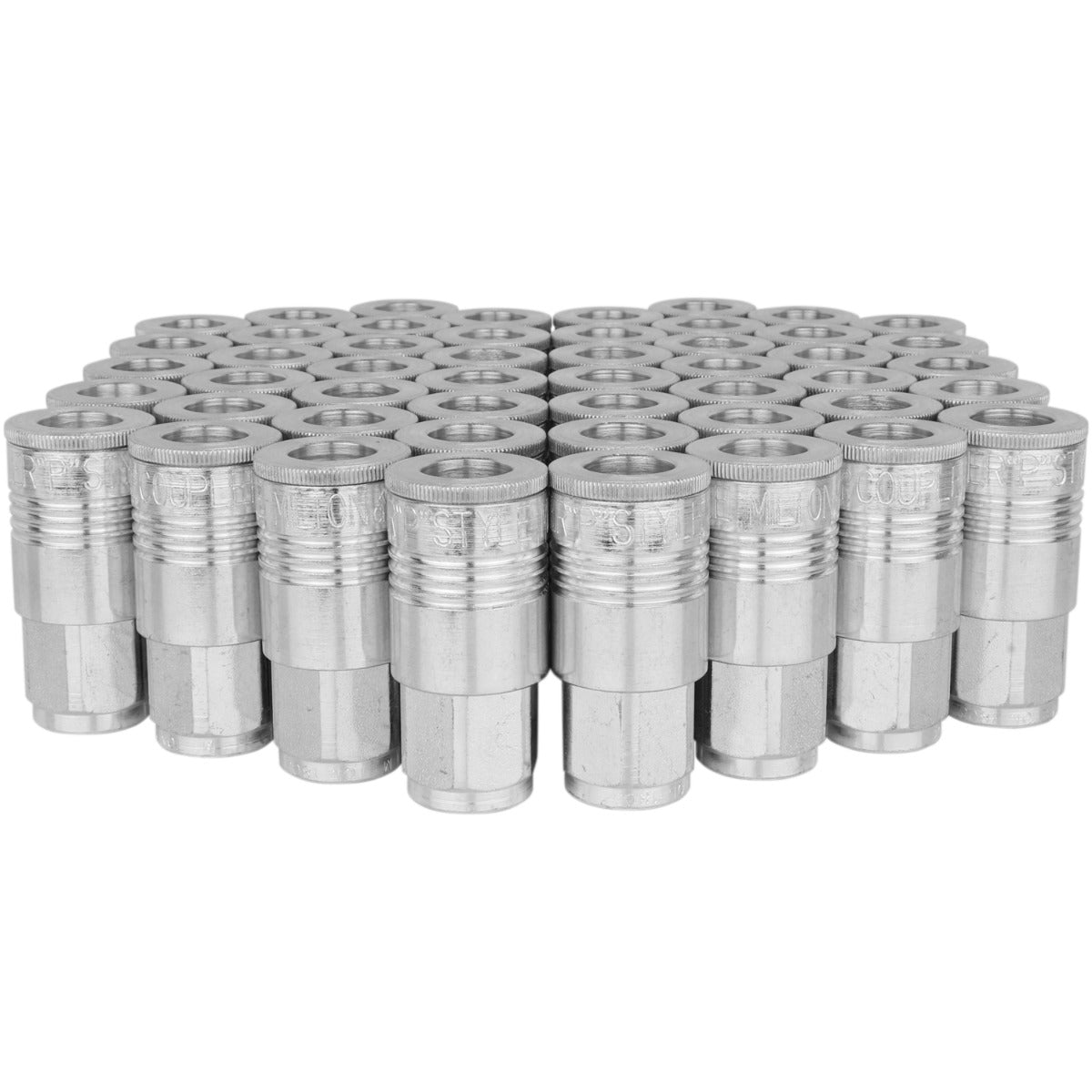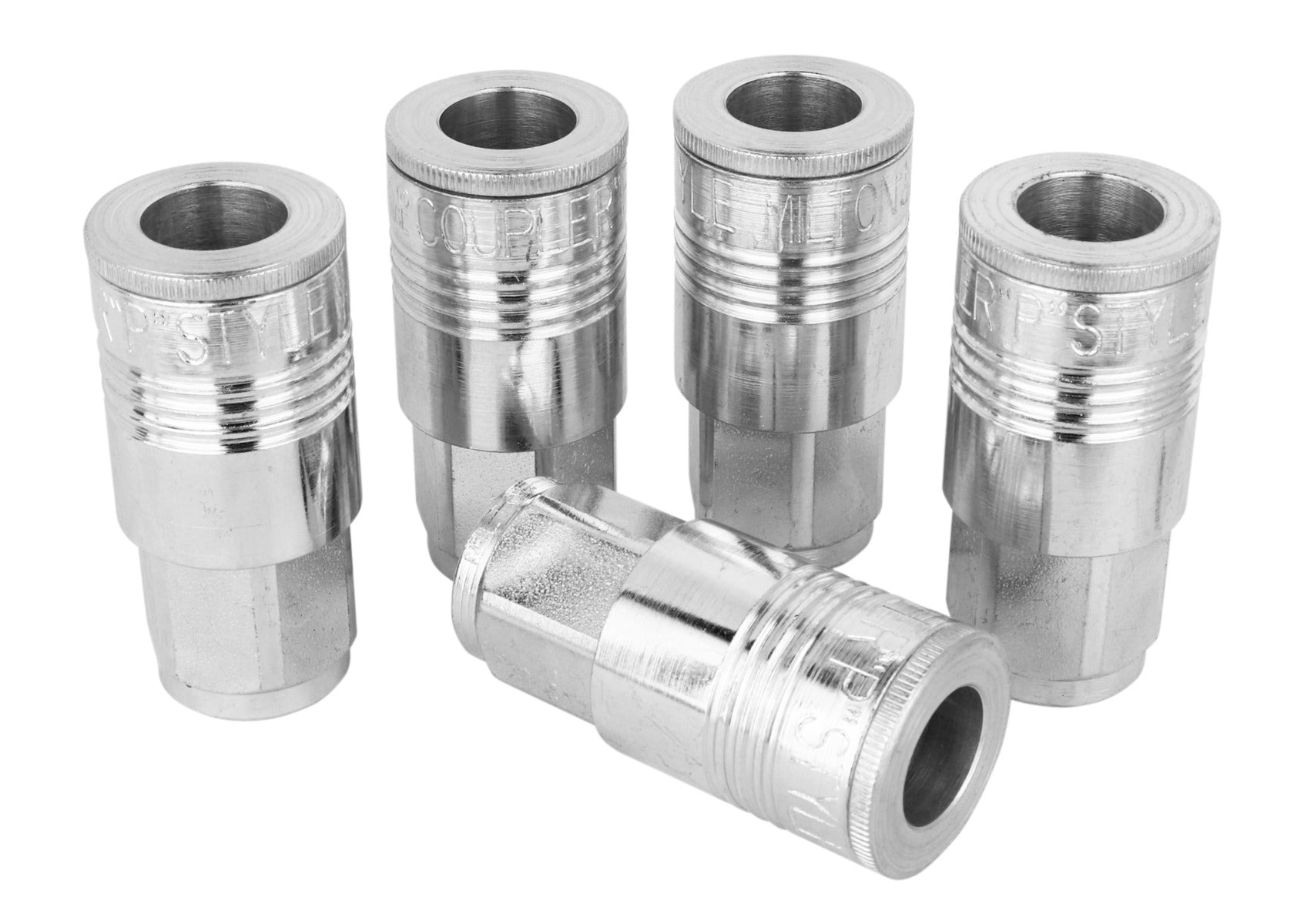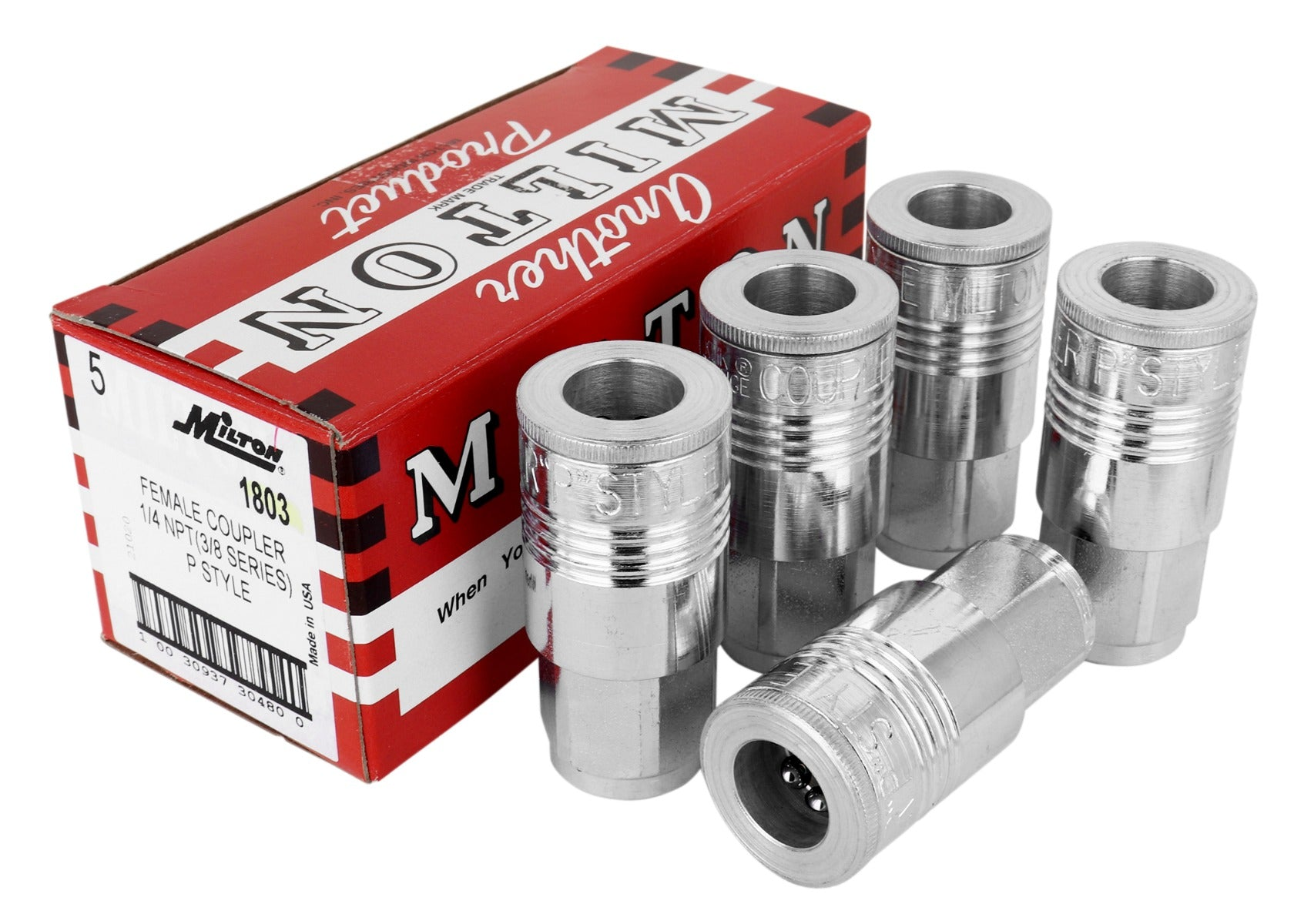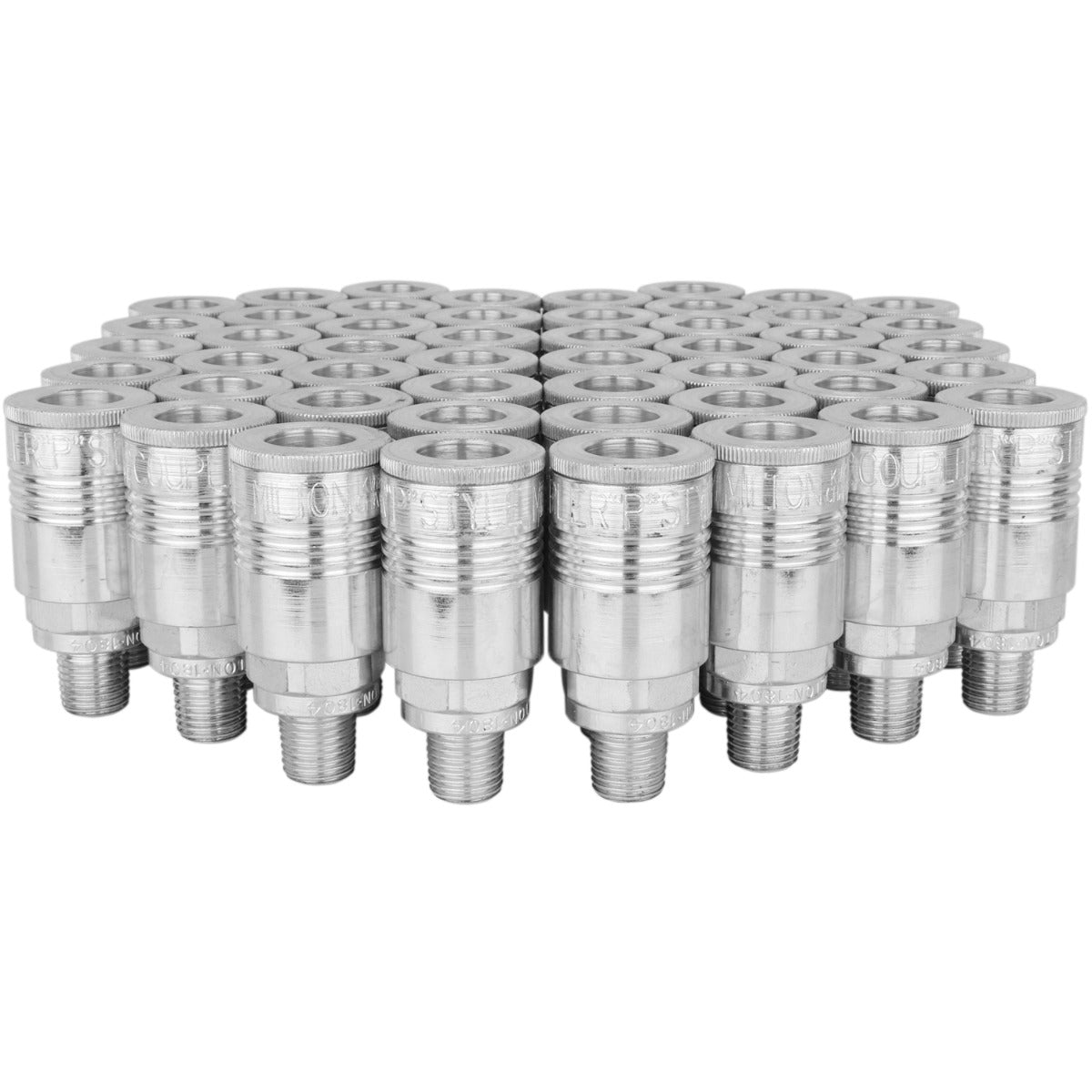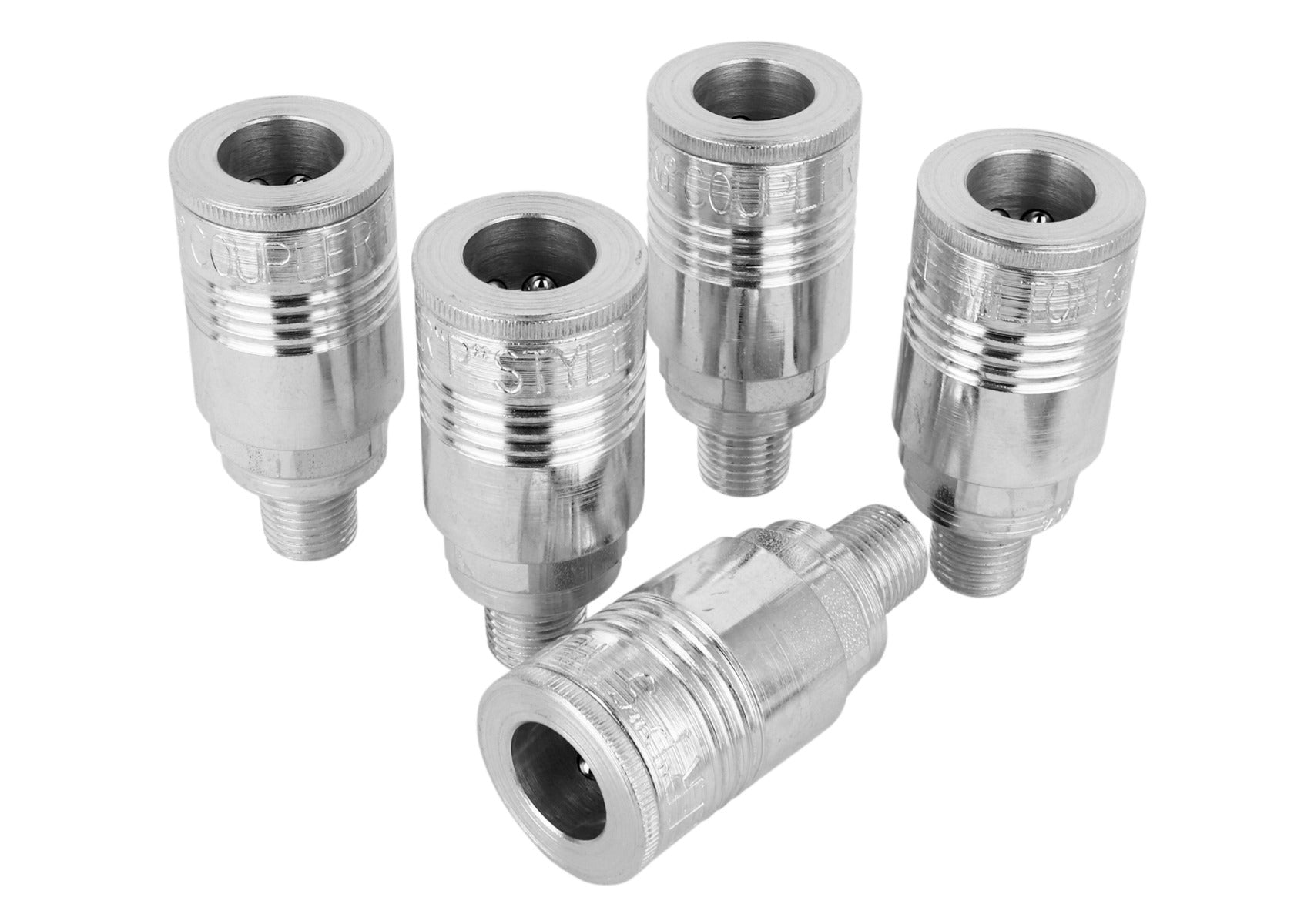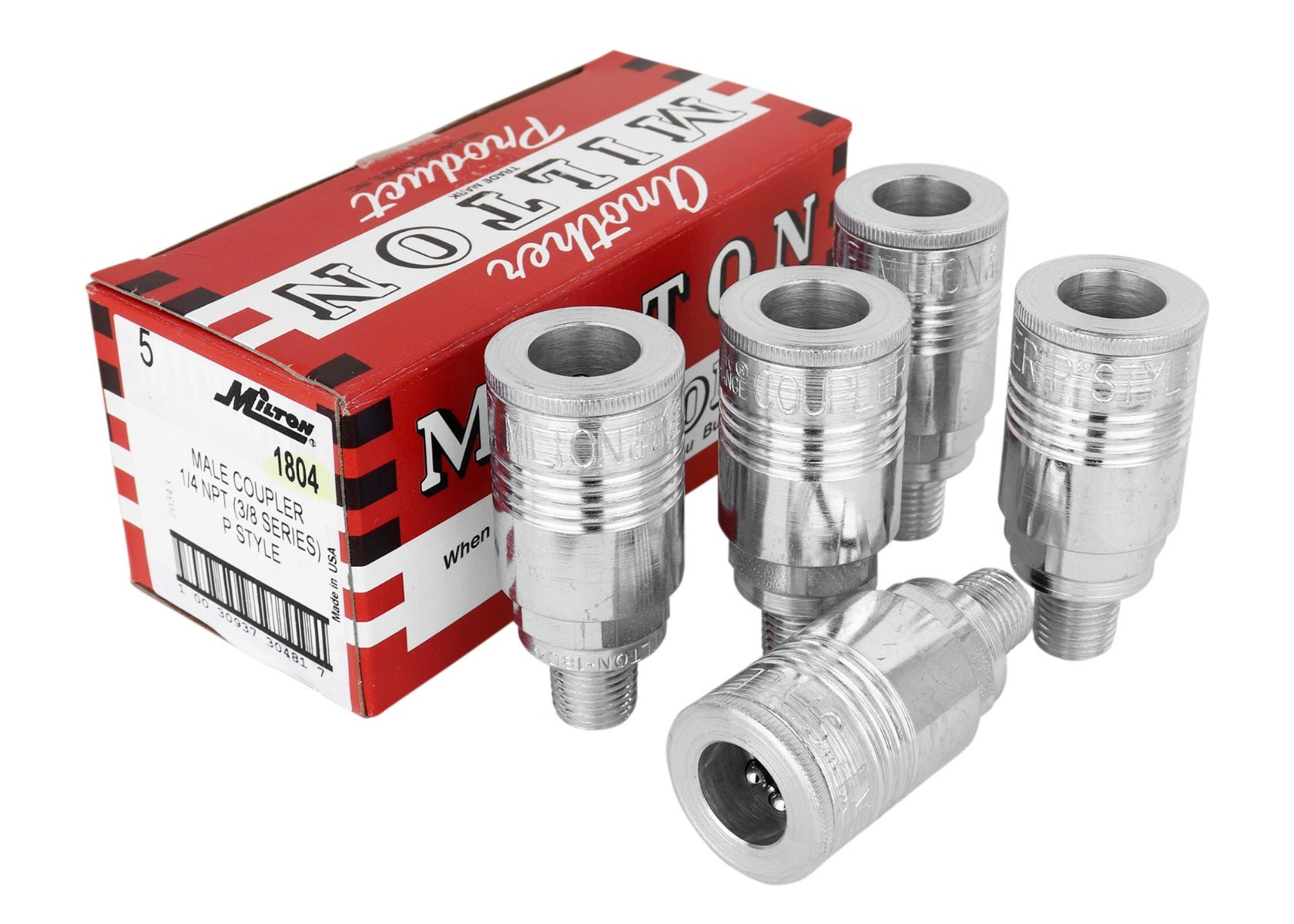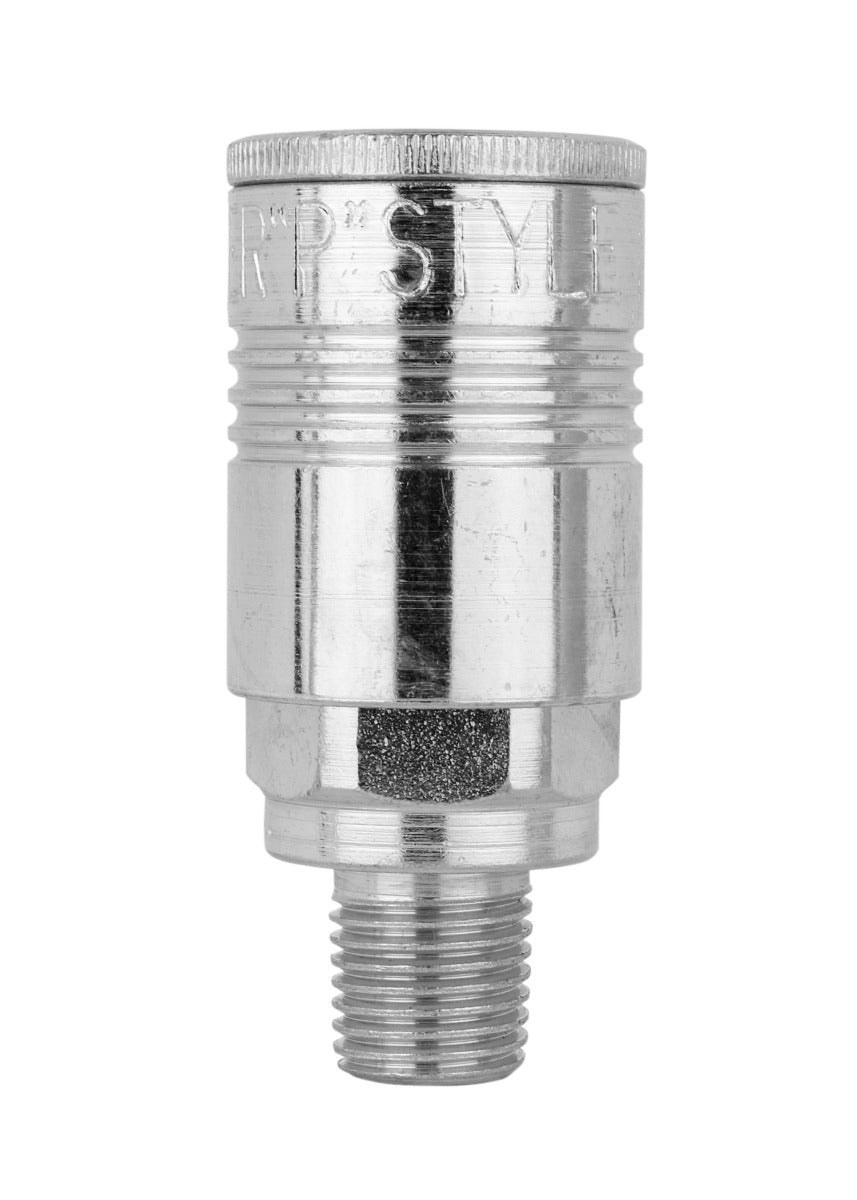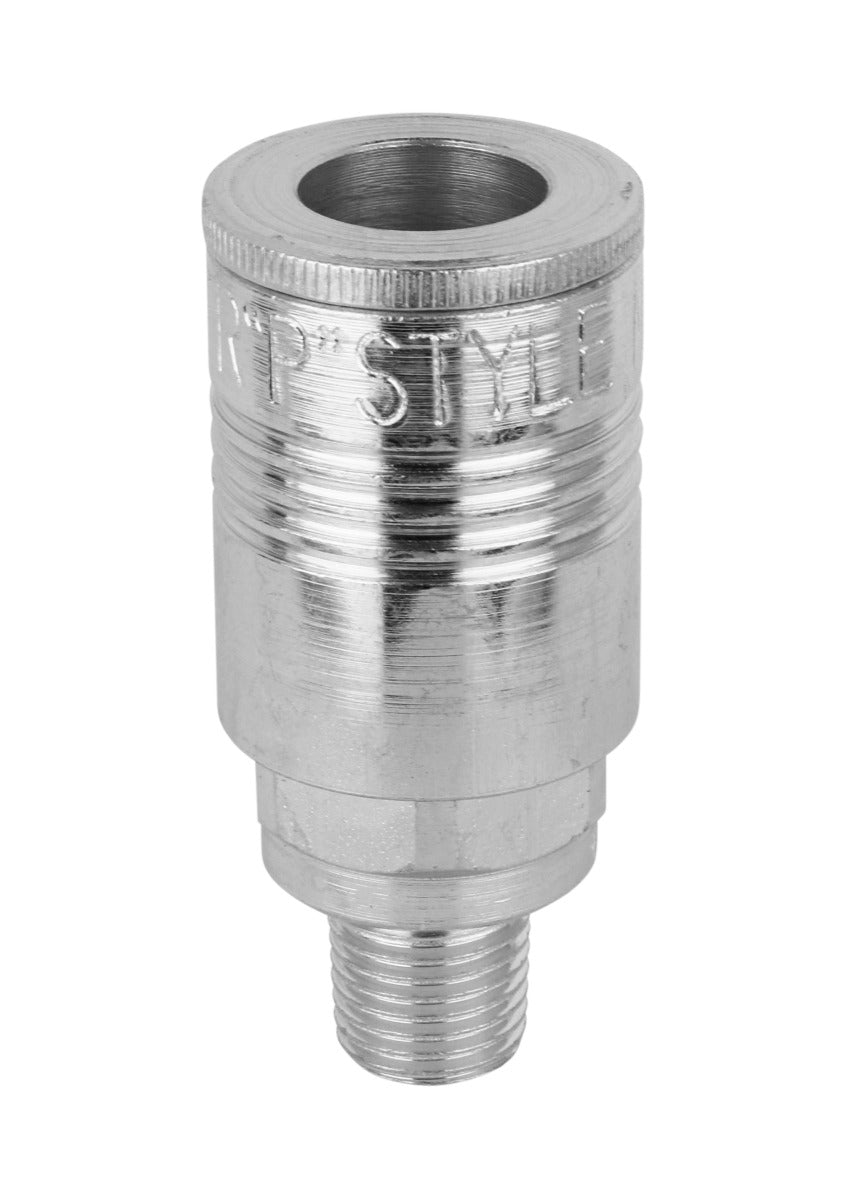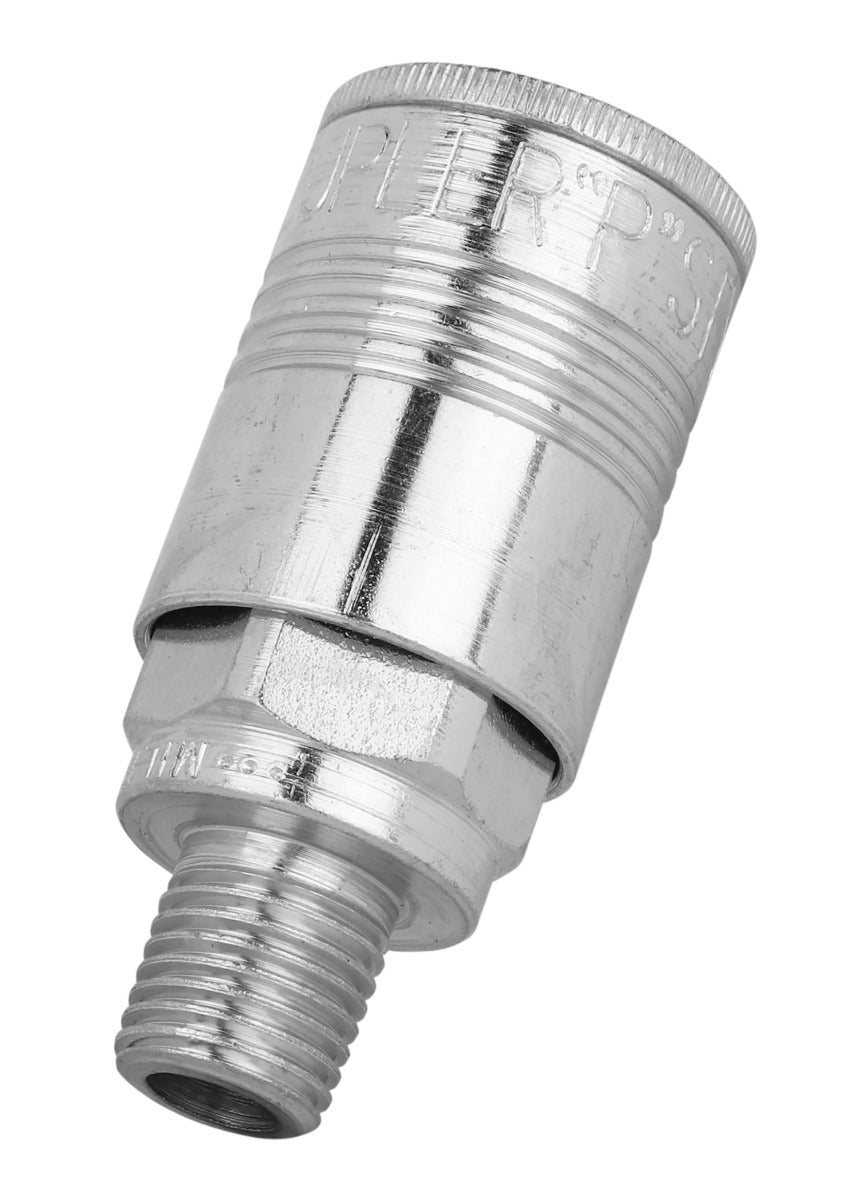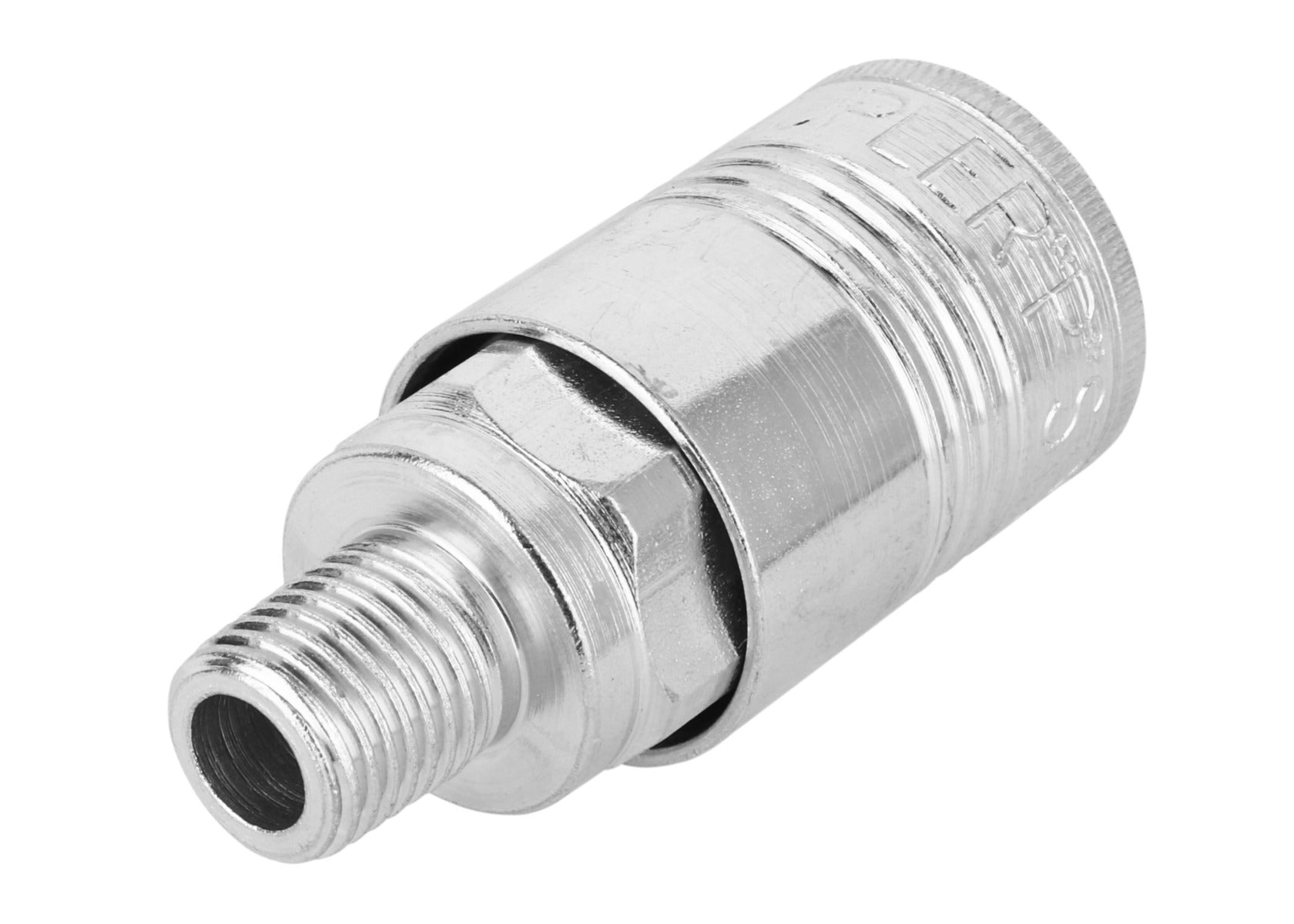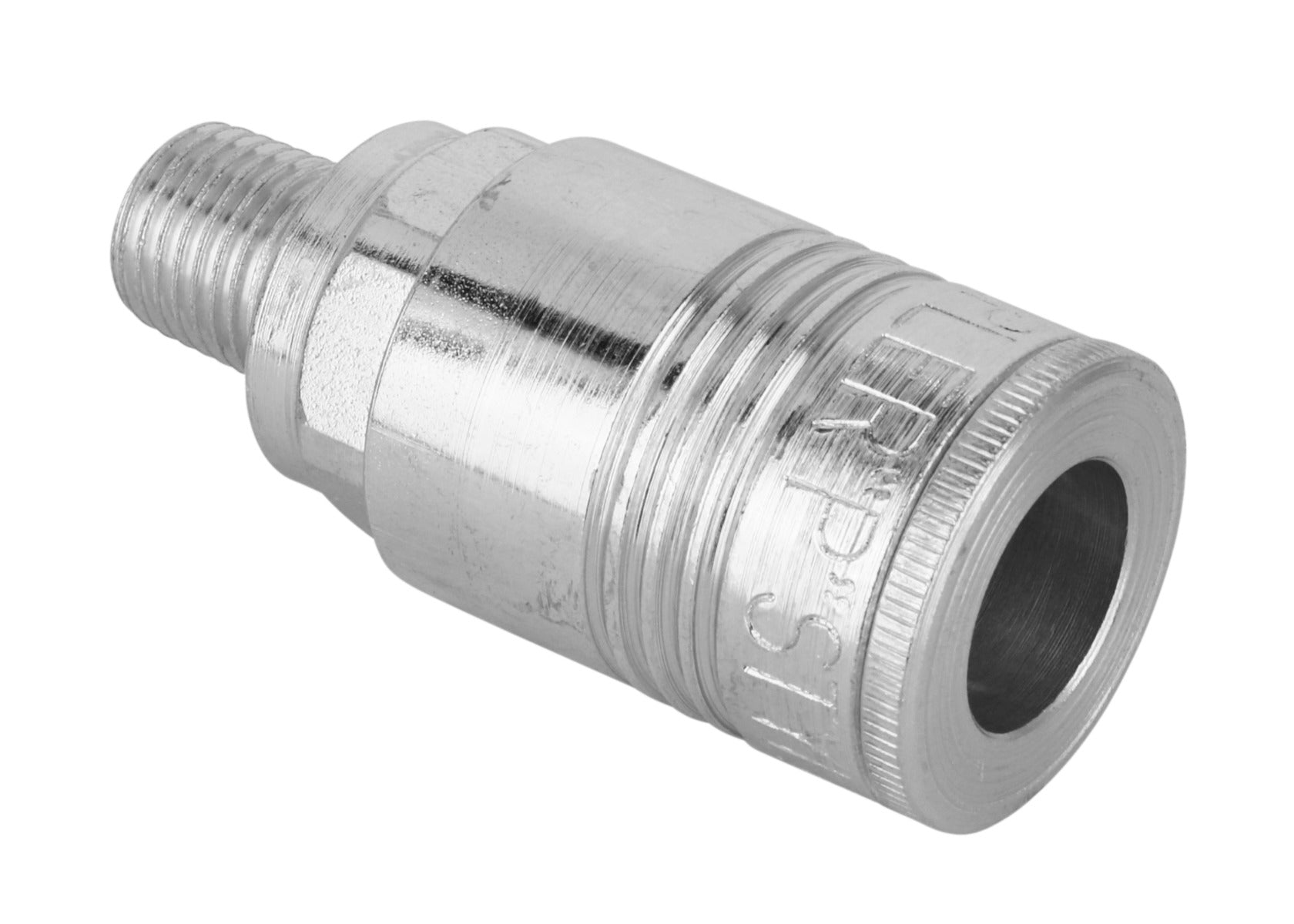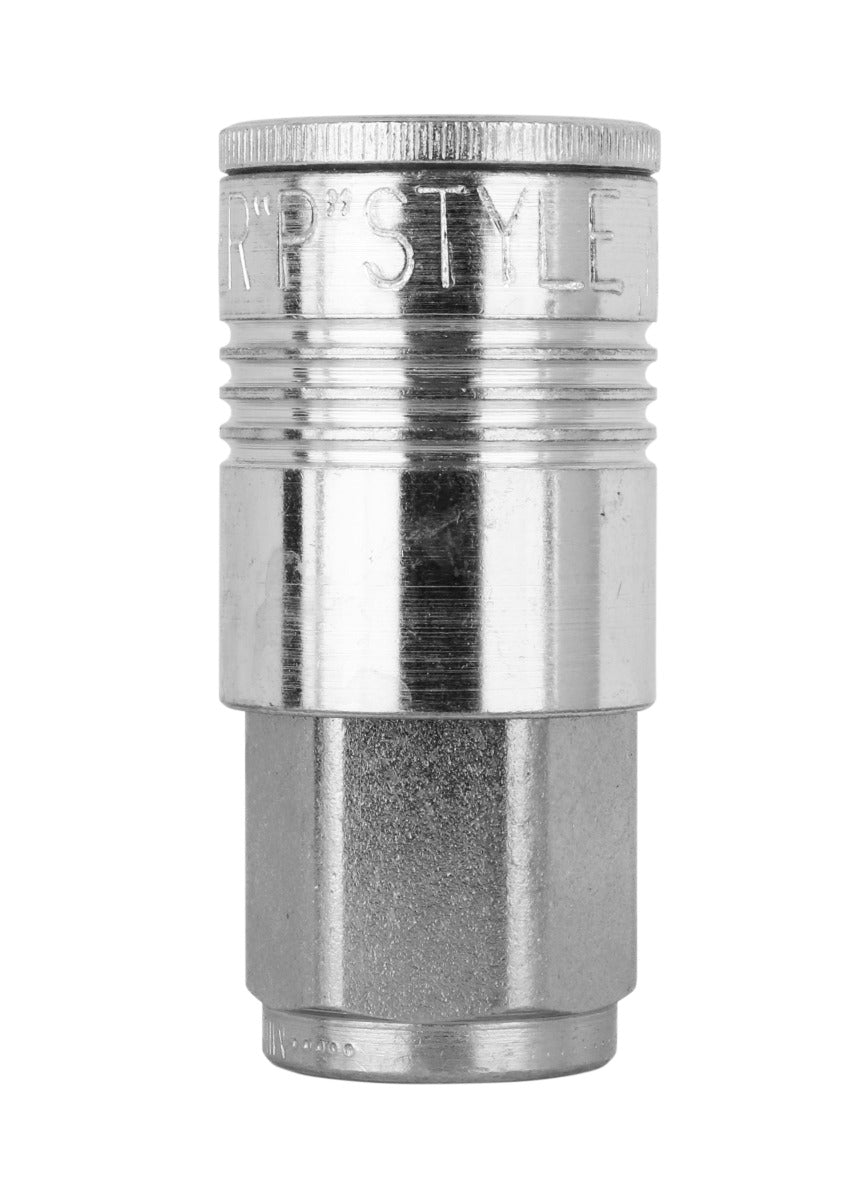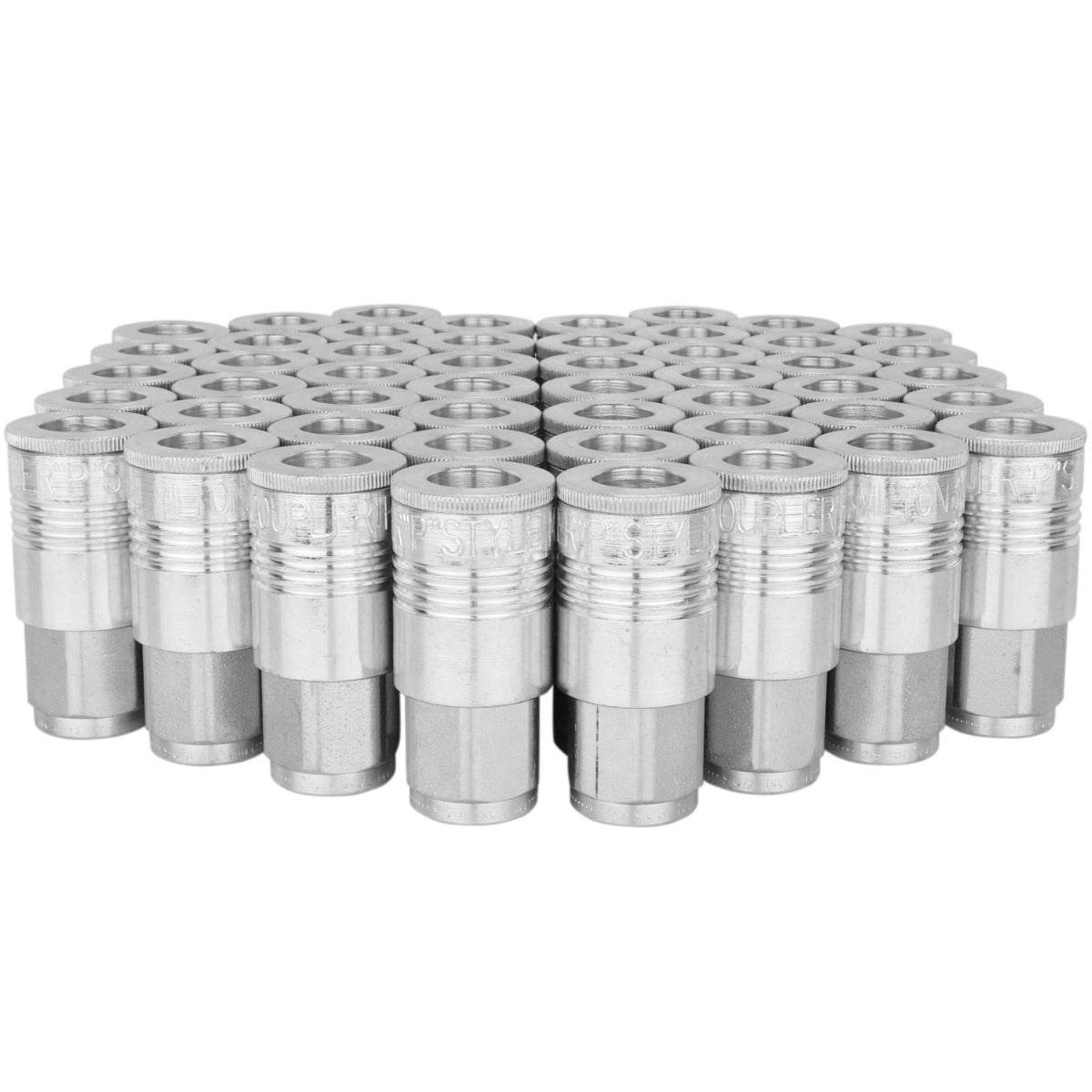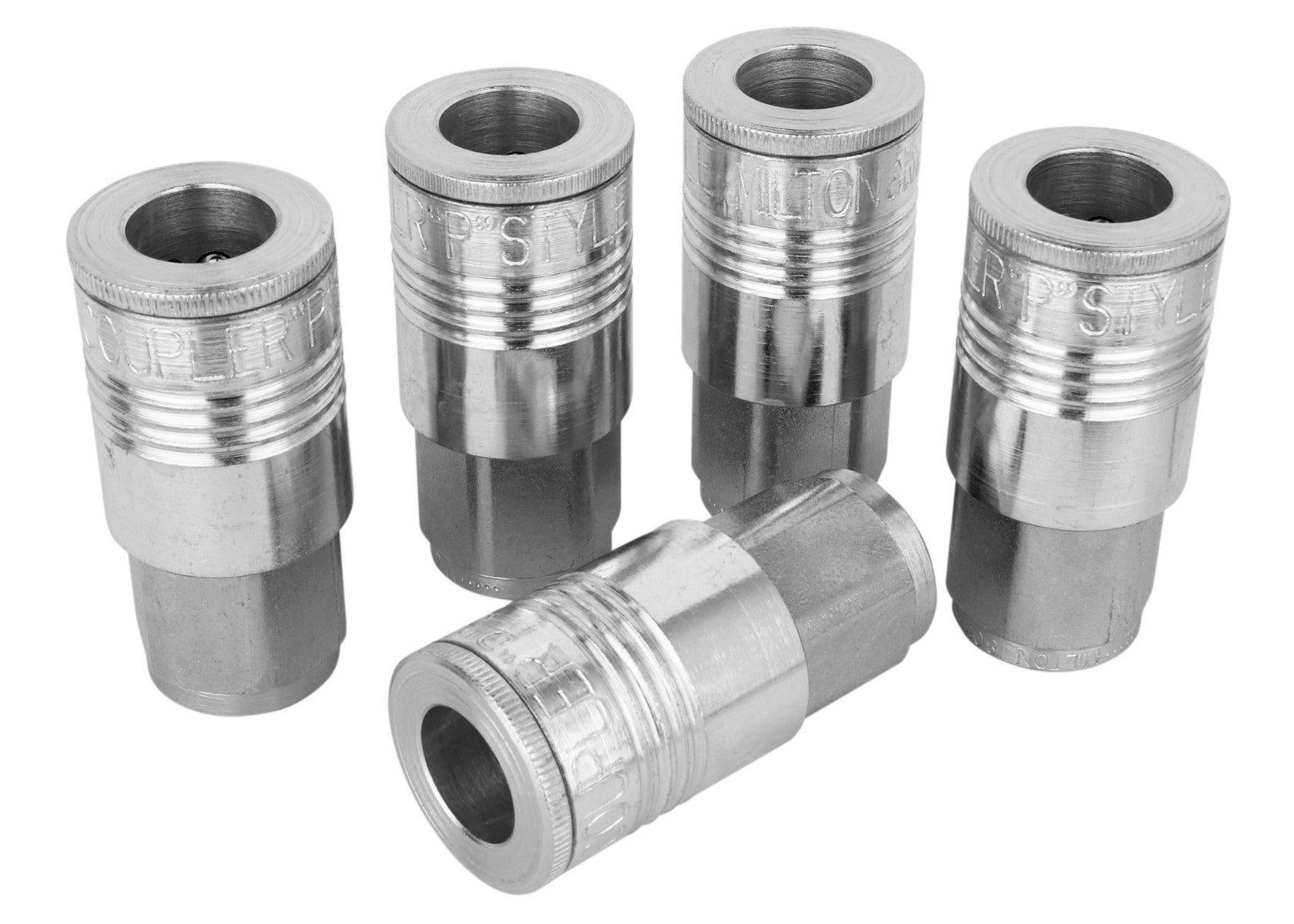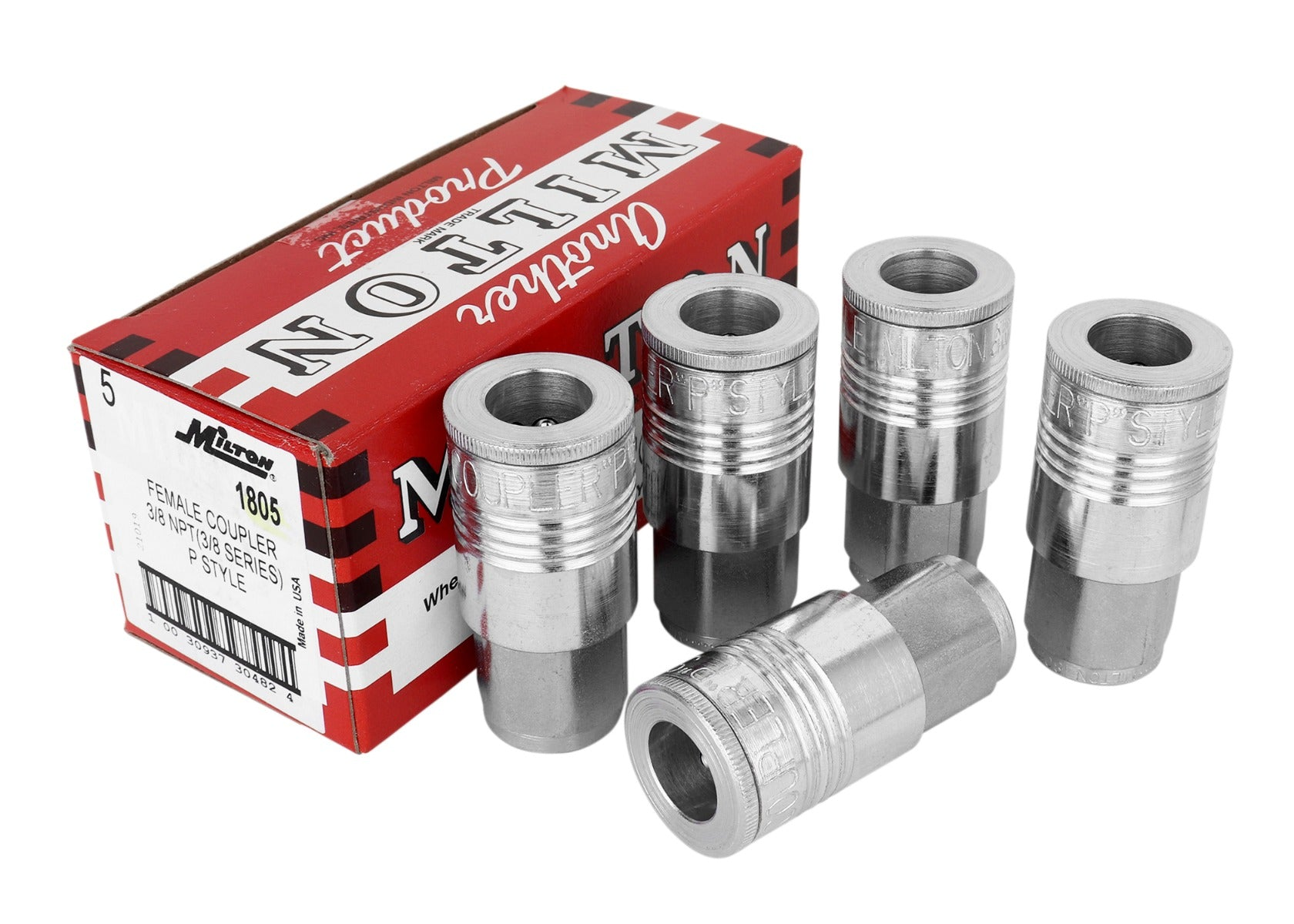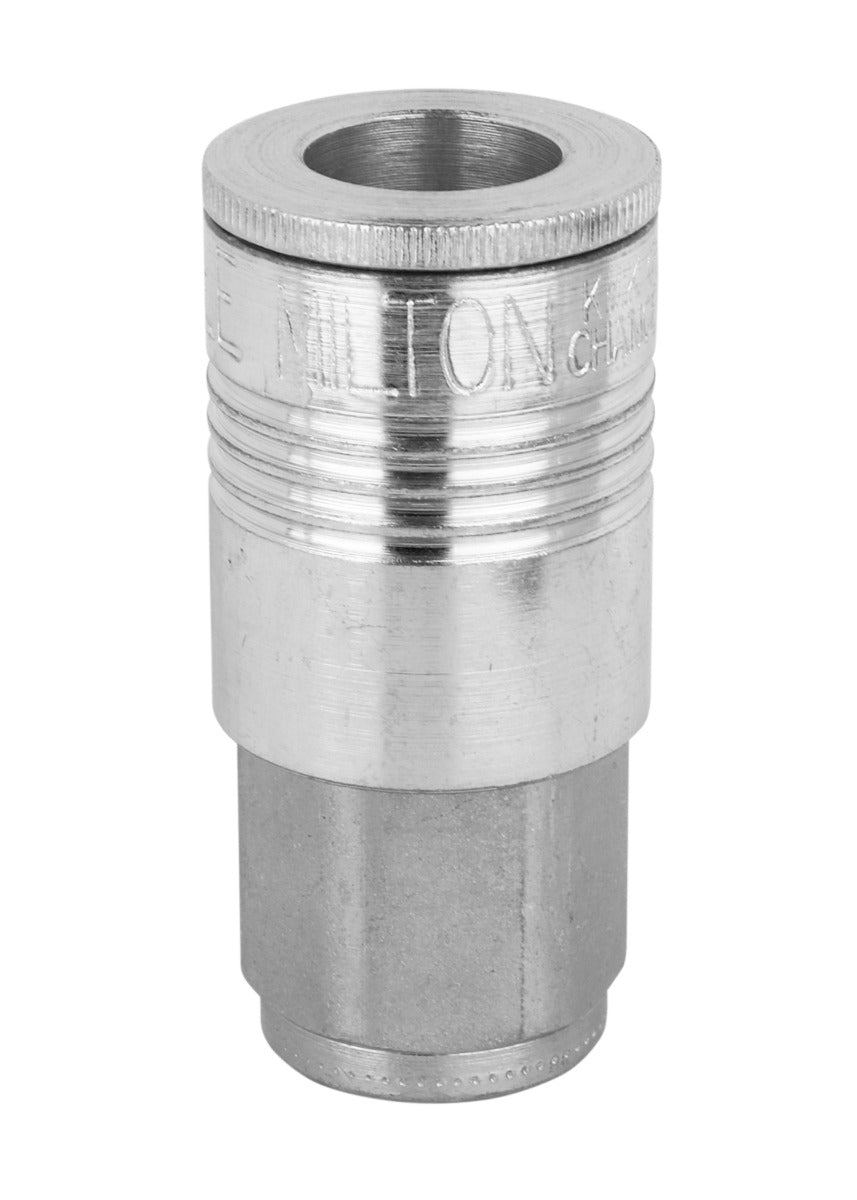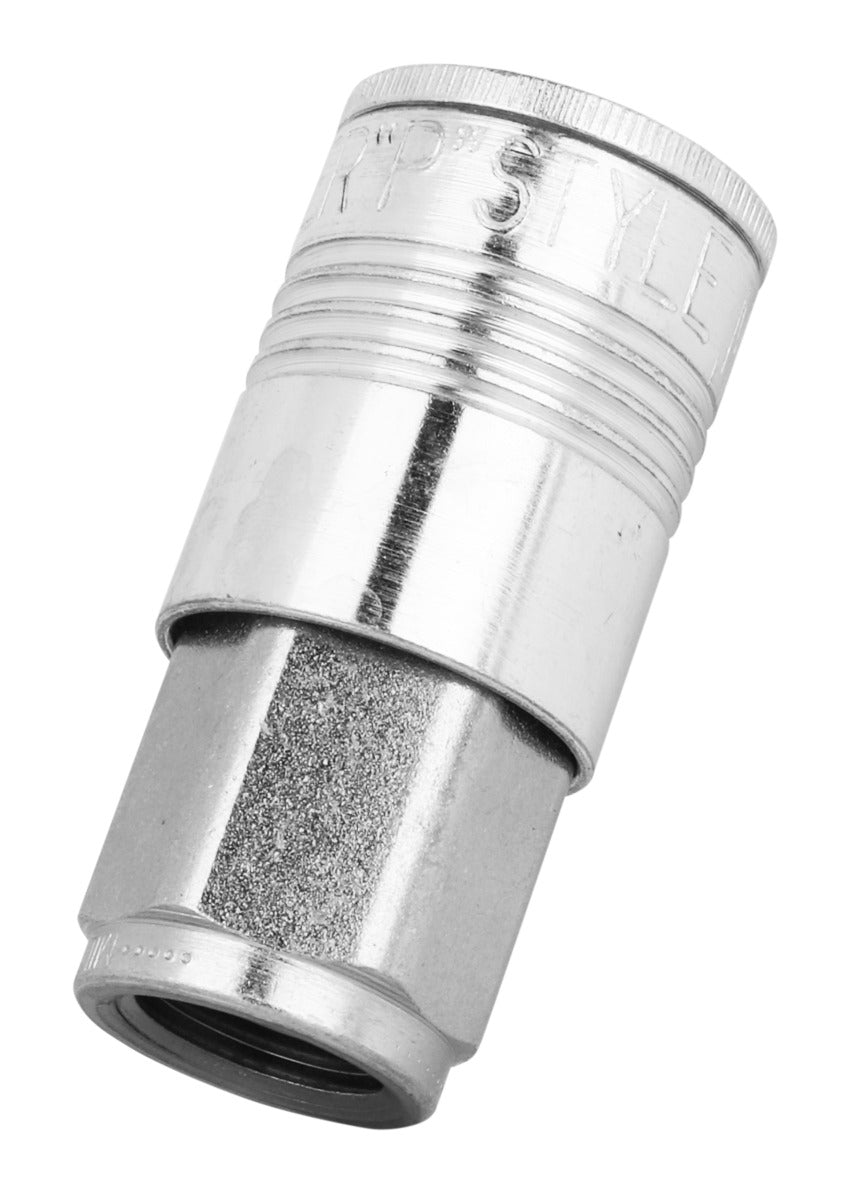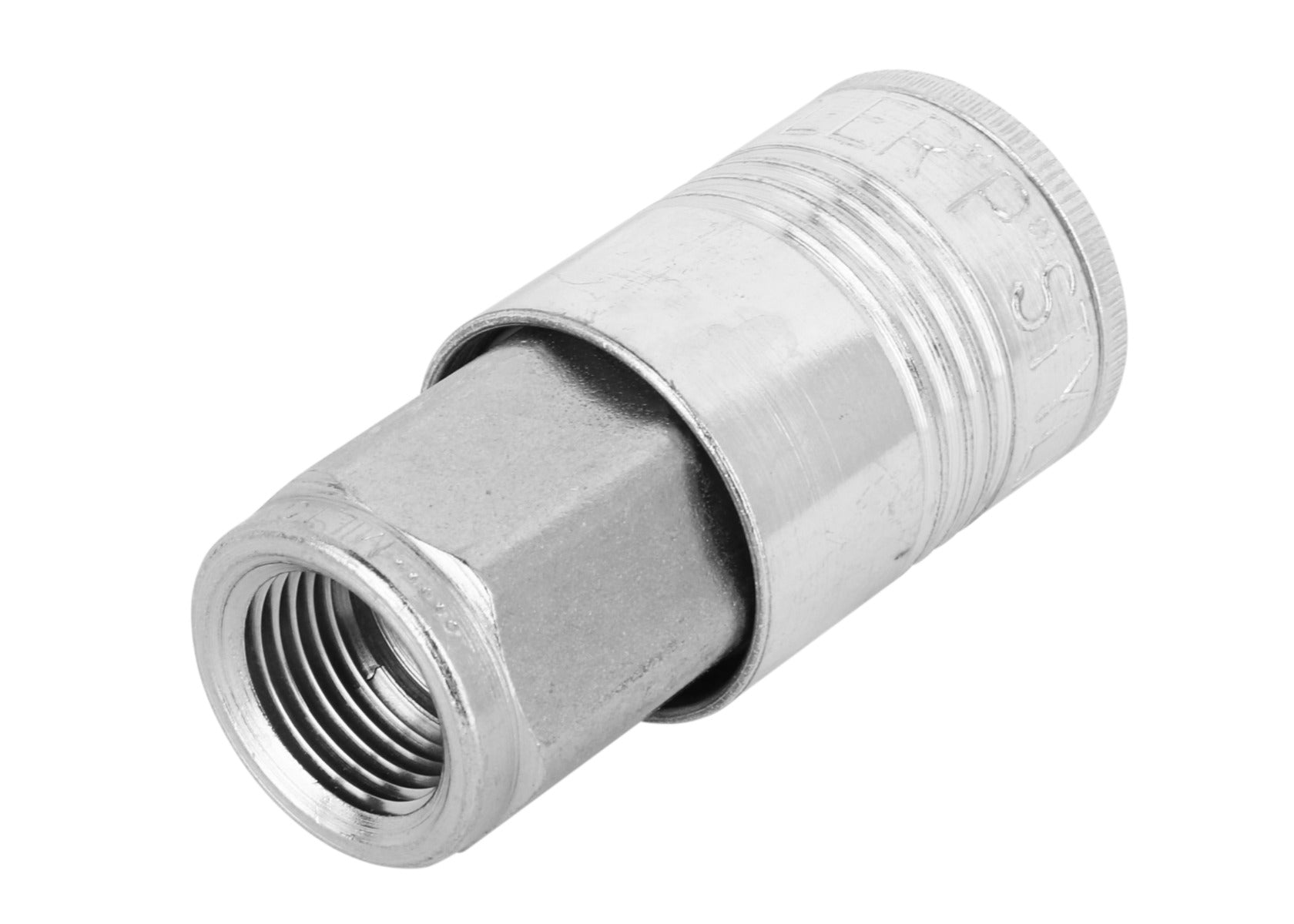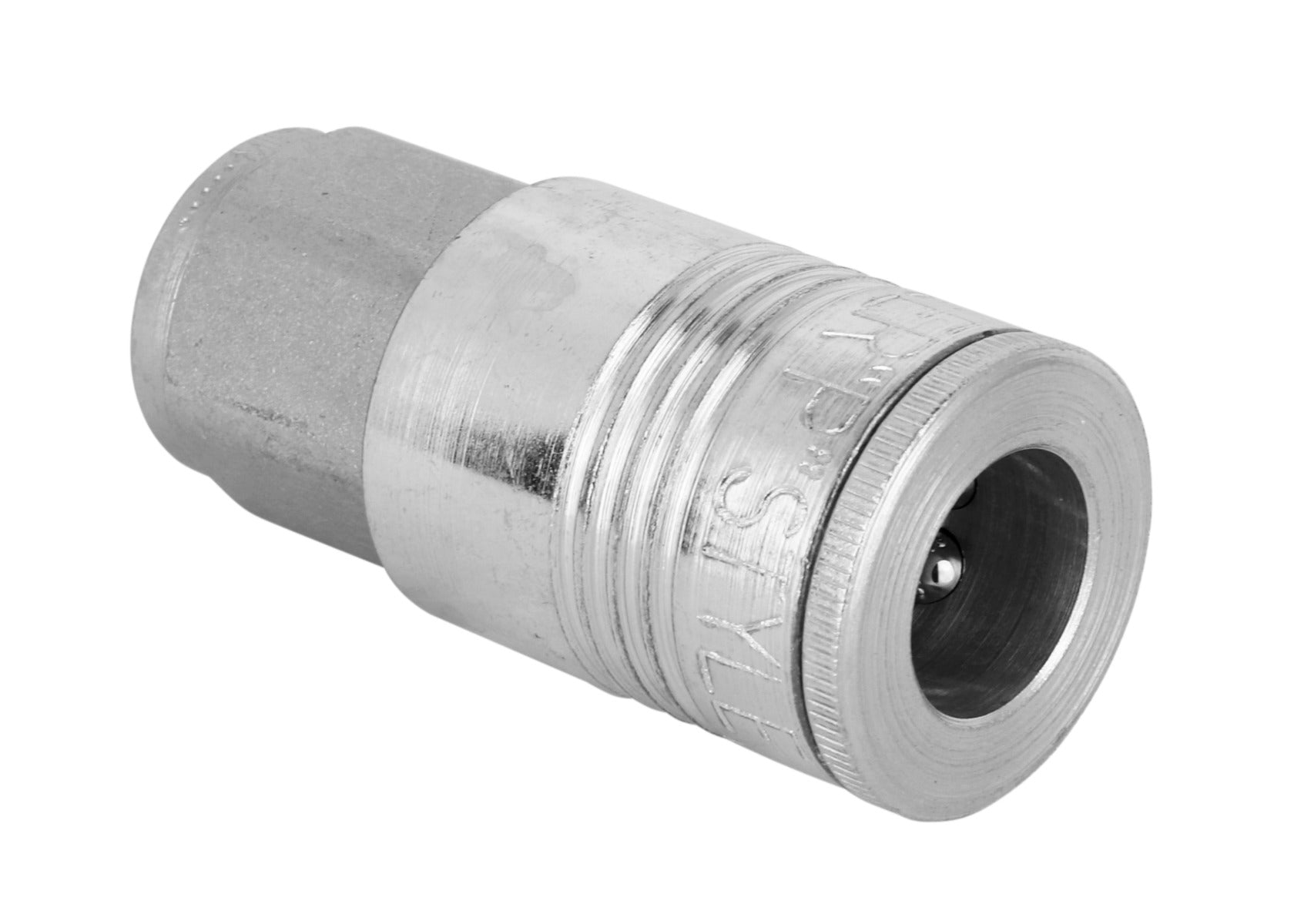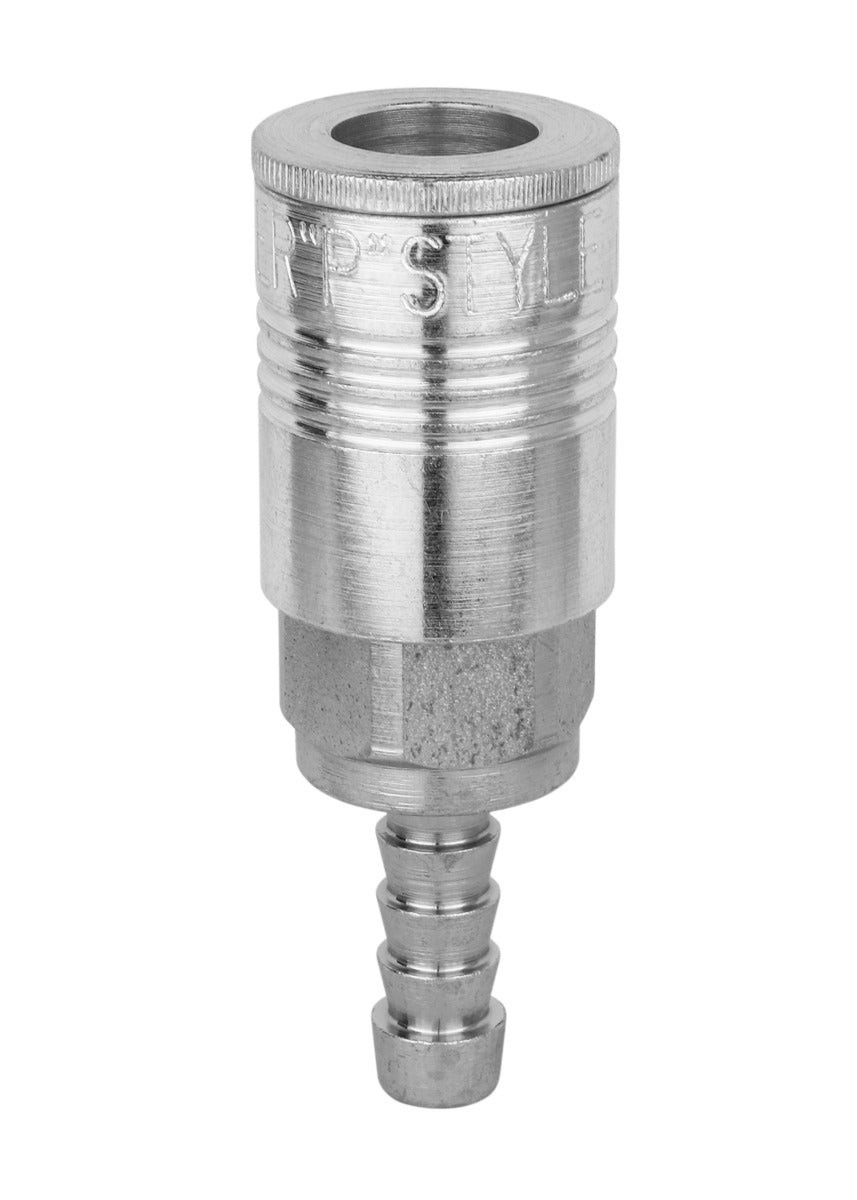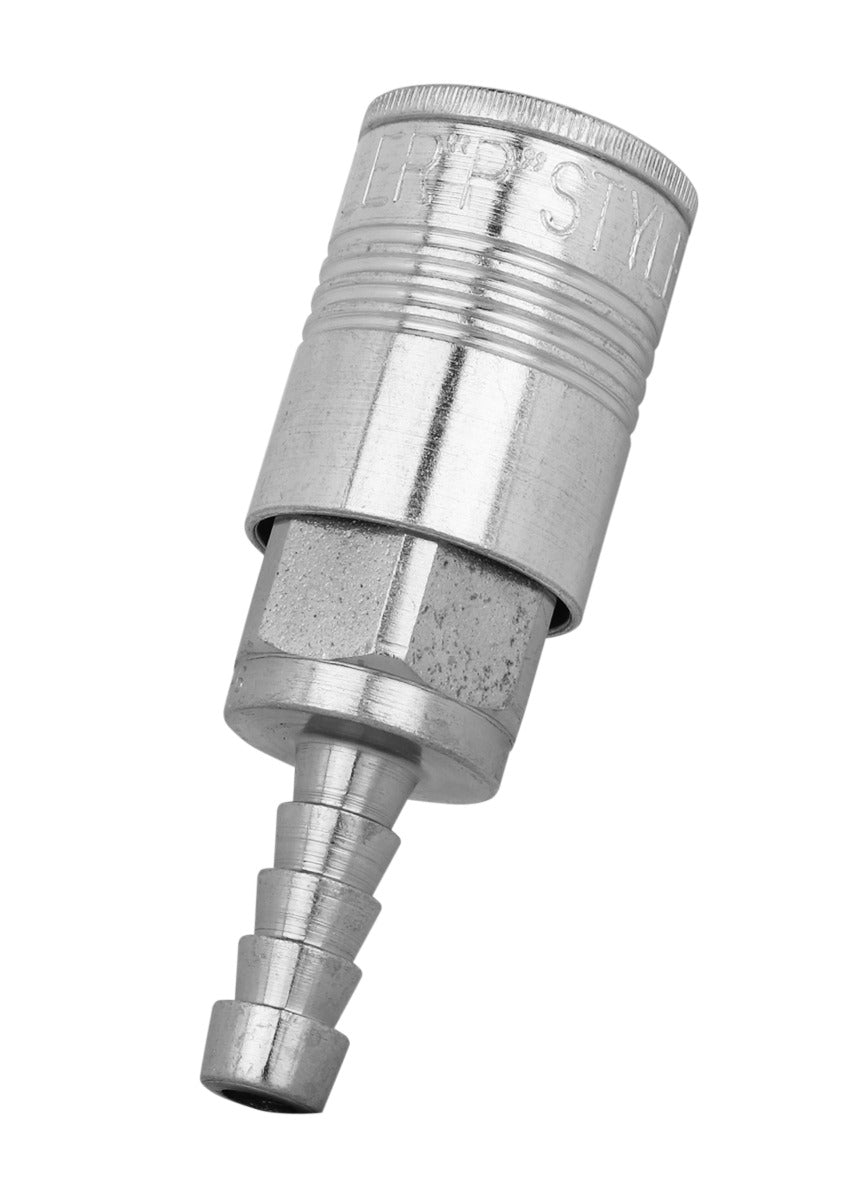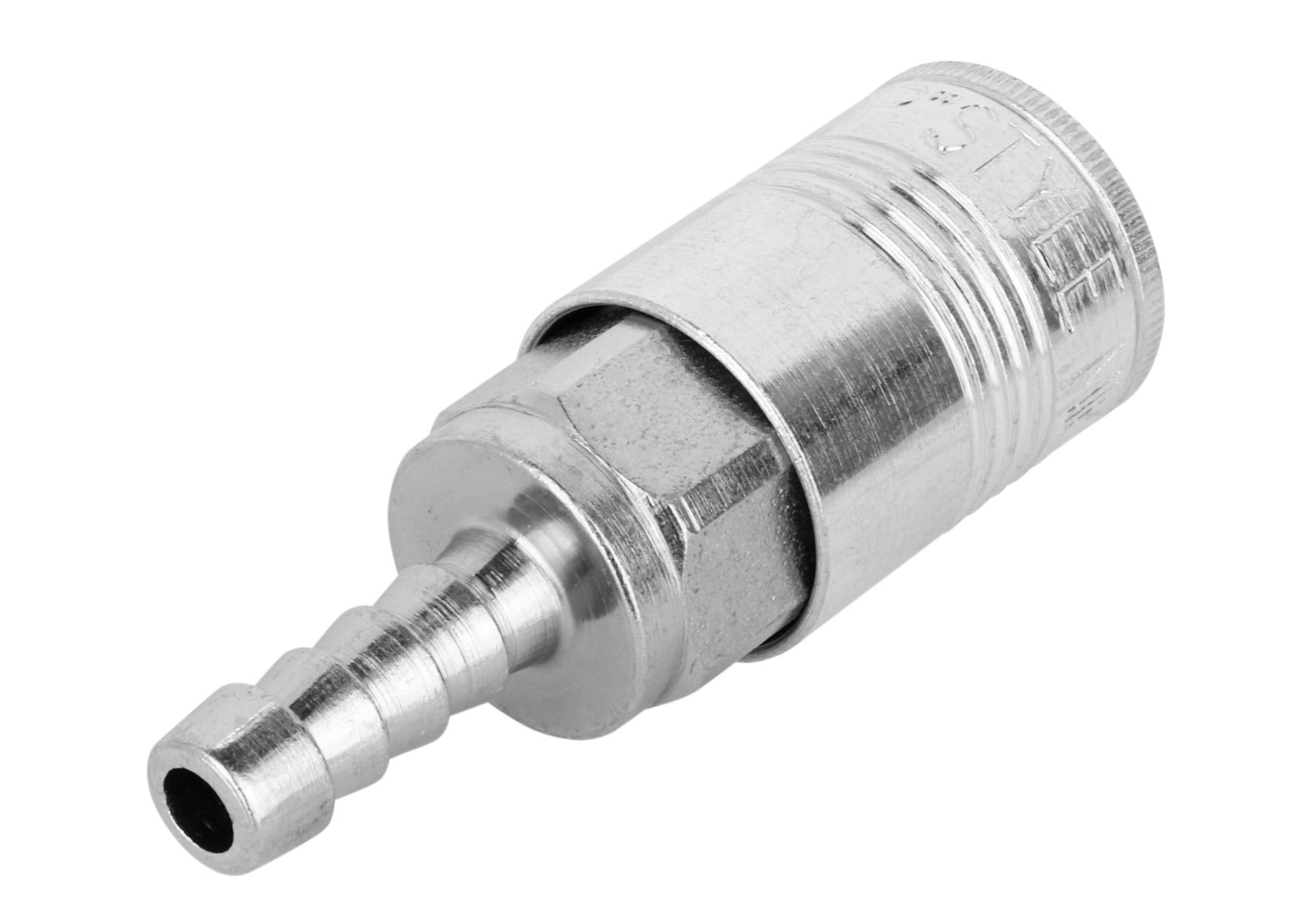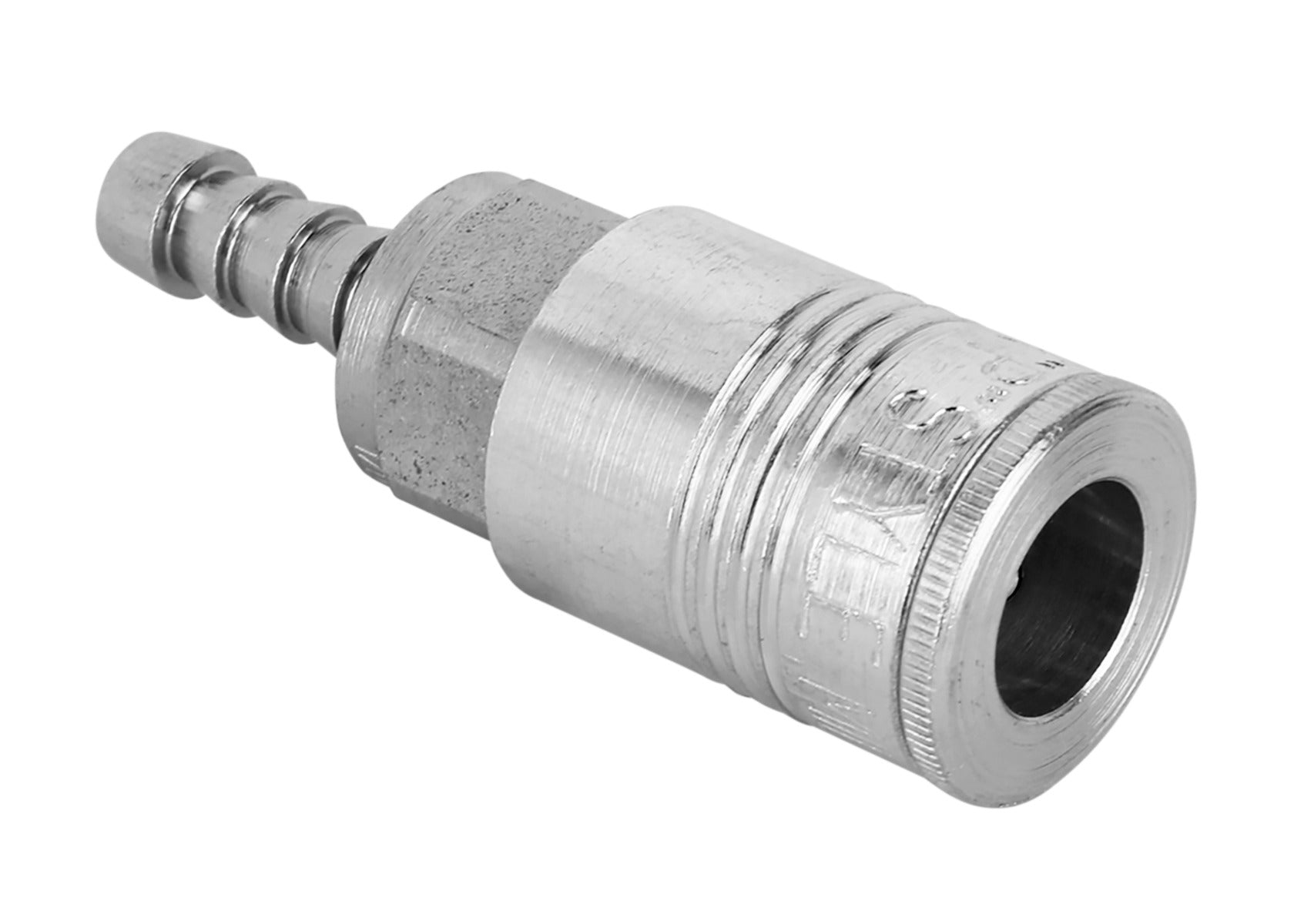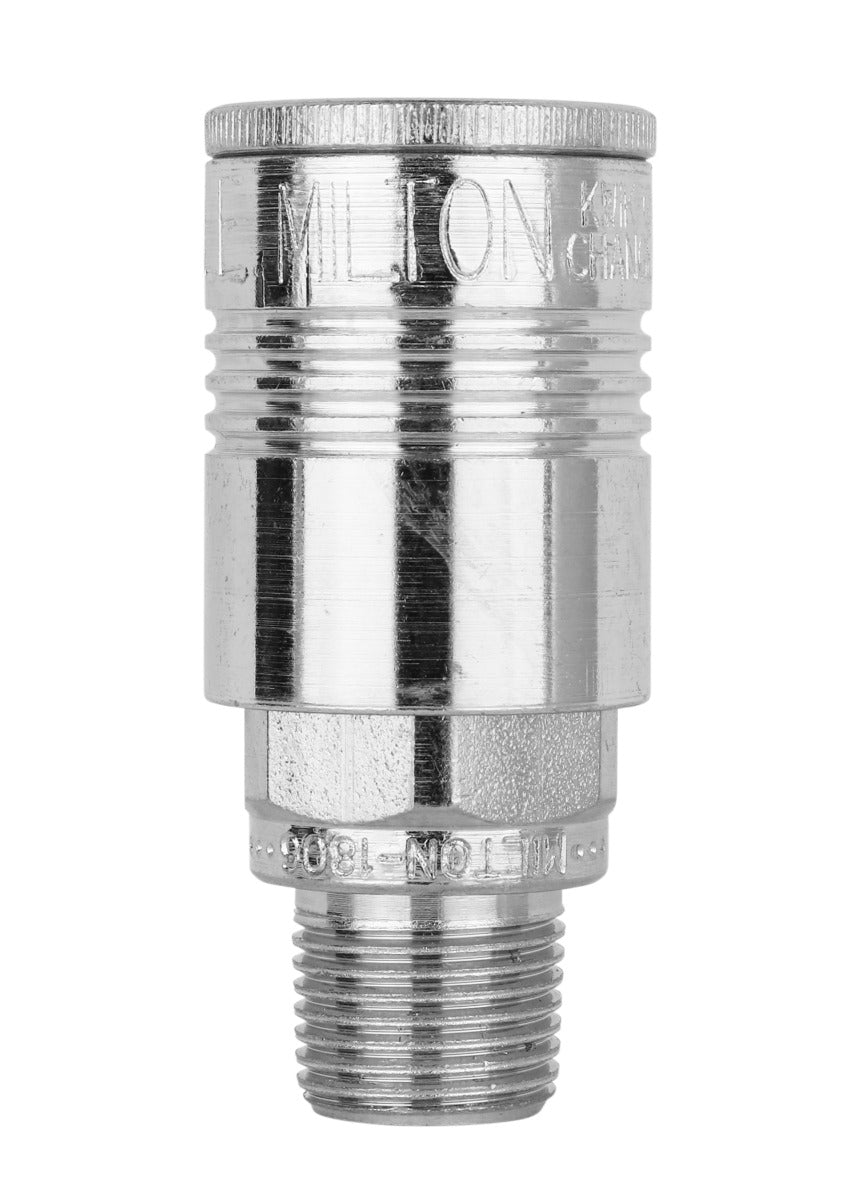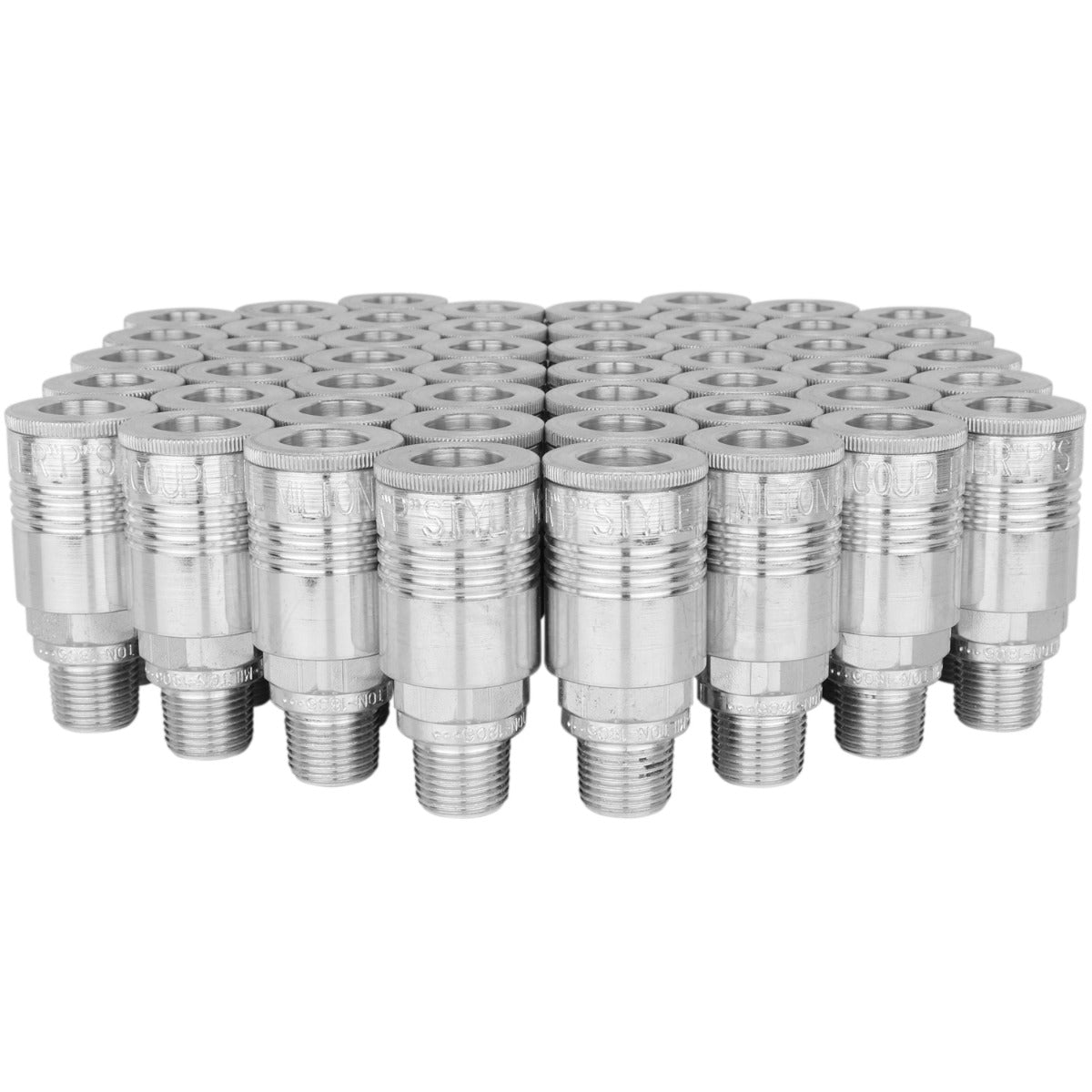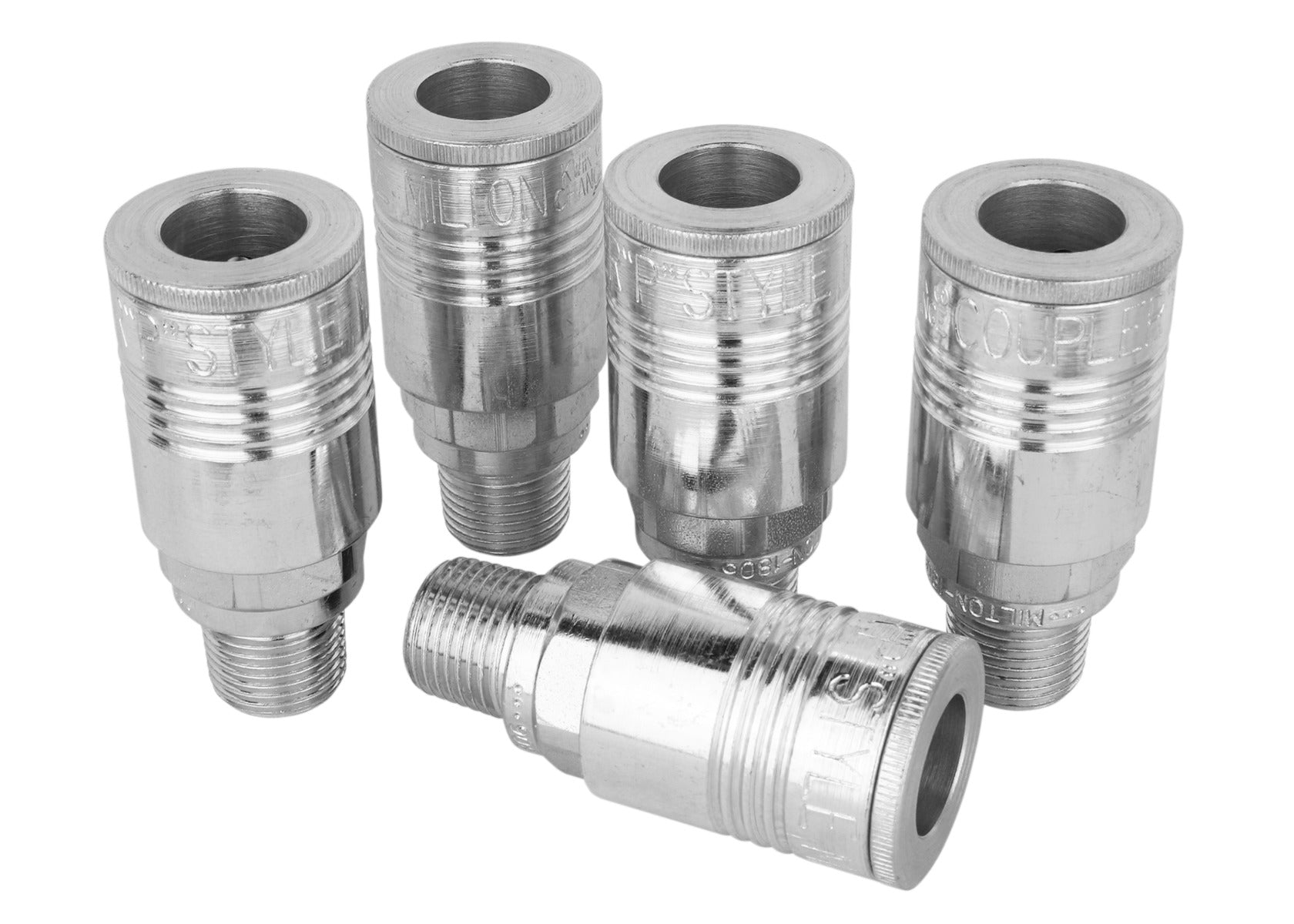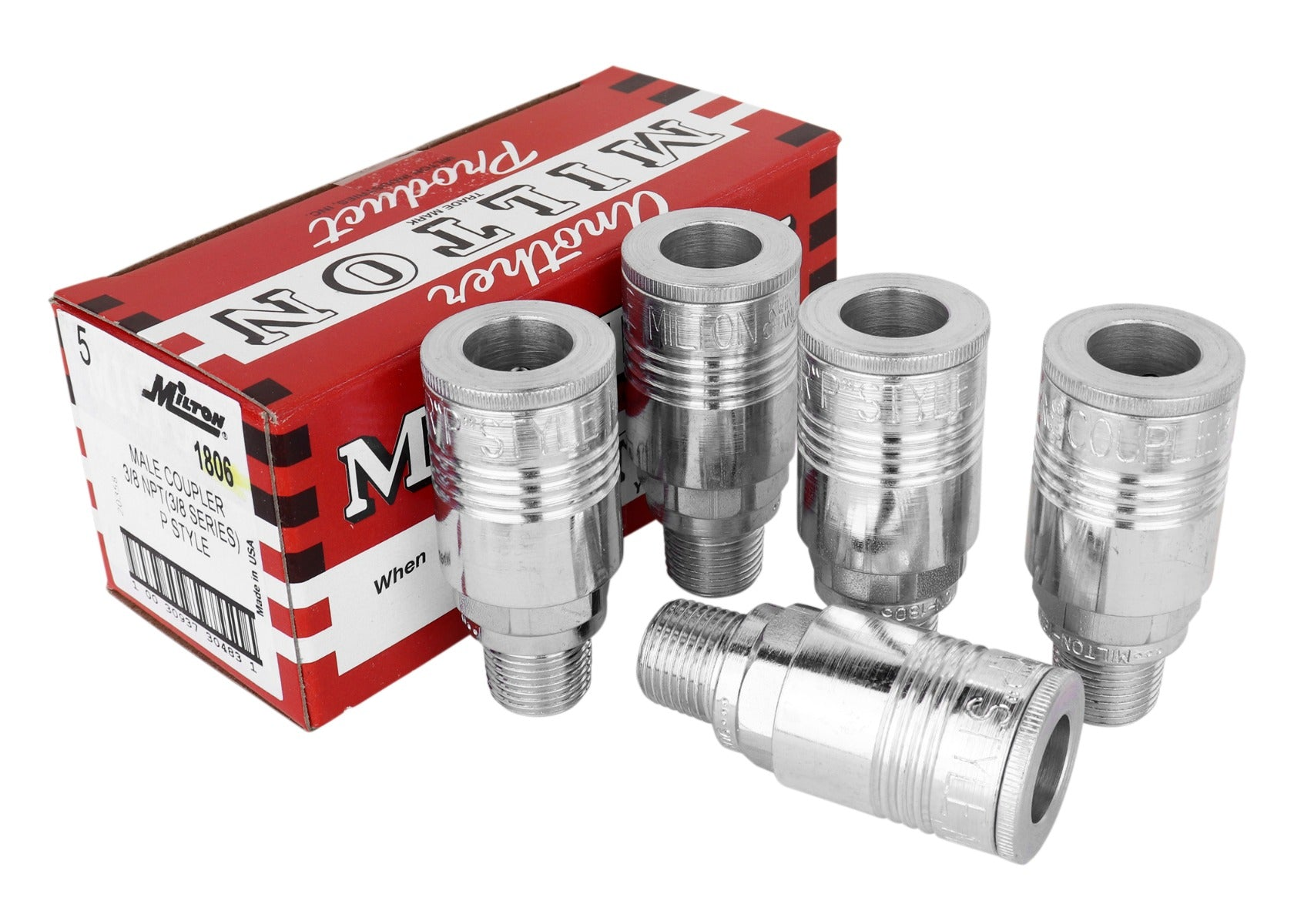Save:# P-Style Coupler

Milton Industries Inc.®

Part #: S-1803W
UPC Code: 30937018038
UPC Code: 30937018038
UPC Code: 30937306548
UPC Code: 30937304803
UPC Code: 30937306555
UPC Code: 30937304810
UPC Code: 30937313980
UPC Code: 30937313980
UPC Code: 30937018052
UPC Code: 30937306562
UPC Code: 30937304827
UPC Code: 30937018052
UPC Code: 30937018069
UPC Code: 30937018069
UPC Code: 30937306579
UPC Code: 30937304834
Number of Items: 1
Number of Items: 5
Number of Items: 50
Number of Items: 5
Number of Items: 50
Number of Items: 5
Number of Items: 1
Number of Items: 5
Number of Items: 5
Number of Items: 50
Number of Items: 5
Number of Items: 1
Number of Items: 1
Number of Items: 5
Number of Items: 50
Number of Items: 5
• Maximum of 300 pounds per square inch
• Maximum air flow of 68 standard cubic feet per minute
• Maximum temperature of 250 degrees Fahrenheit
• Maximum of 300 pounds per square inch
• Maximum air flow of 68 standard cubic feet per minute
• Maximum temperature of 250 degrees Fahrenheit
• Maximum of 300 pounds per square inch
• Maximum air flow of 68 standard cubic feet per minute
• Maximum temperature of 250 degrees Fahrenheit
• Maximum of 300 pounds per square inch
• Maximum air flow of 68 standard cubic feet per minute
• Maximum temperature of 250 degrees Fahrenheit
• Maximum of 300 pounds per square inch
• Maximum air flow of 68 standard cubic feet per minute
• Maximum temperature of 250 degrees Fahrenheit
• Maximum of 300 pounds per square inch
• Maximum air flow of 68 standard cubic feet per minute
• Maximum temperature of 250 degrees Fahrenheit
• Maximum of 300 pounds per square inch
• Maximum air flow of 68 standard cubic feet per minute
• Maximum temperature of 250 degrees Fahrenheit
• Maximum of 300 pounds per square inch
• Maximum air flow of 68 standard cubic feet per minute
• Maximum temperature of 250 degrees Fahrenheit
• Maximum of 300 pounds per square inch
• Maximum air flow of 68 standard cubic feet per minute
• Maximum temperature of 250 degrees Fahrenheit
• Maximum of 300 pounds per square inch
• Maximum air flow of 68 standard cubic feet per minute
• Maximum temperature of 250 degrees Fahrenheit
• Maximum of 300 pounds per square inch
• Maximum air flow of 68 standard cubic feet per minute
• Maximum temperature of 250 degrees Fahrenheit
• Maximum of 300 pounds per square inch
• Maximum air flow of 68 standard cubic feet per minute
• Maximum temperature of 250 degrees Fahrenheit
• Maximum of 300 pounds per square inch
• Maximum air flow of 68 standard cubic feet per minute
• Maximum temperature of 250 degrees Fahrenheit
• Maximum of 300 pounds per square inch
• Maximum air flow of 68 standard cubic feet per minute
• Maximum temperature of 250 degrees Fahrenheit
• Maximum of 300 pounds per square inch
• Maximum air flow of 68 standard cubic feet per minute
• Maximum temperature of 250 degrees Fahrenheit
• Maximum of 300 pounds per square inch
• Maximum air flow of 68 standard cubic feet per minute
• Maximum temperature of 250 degrees Fahrenheit
\$19.30
Save:

### Quantity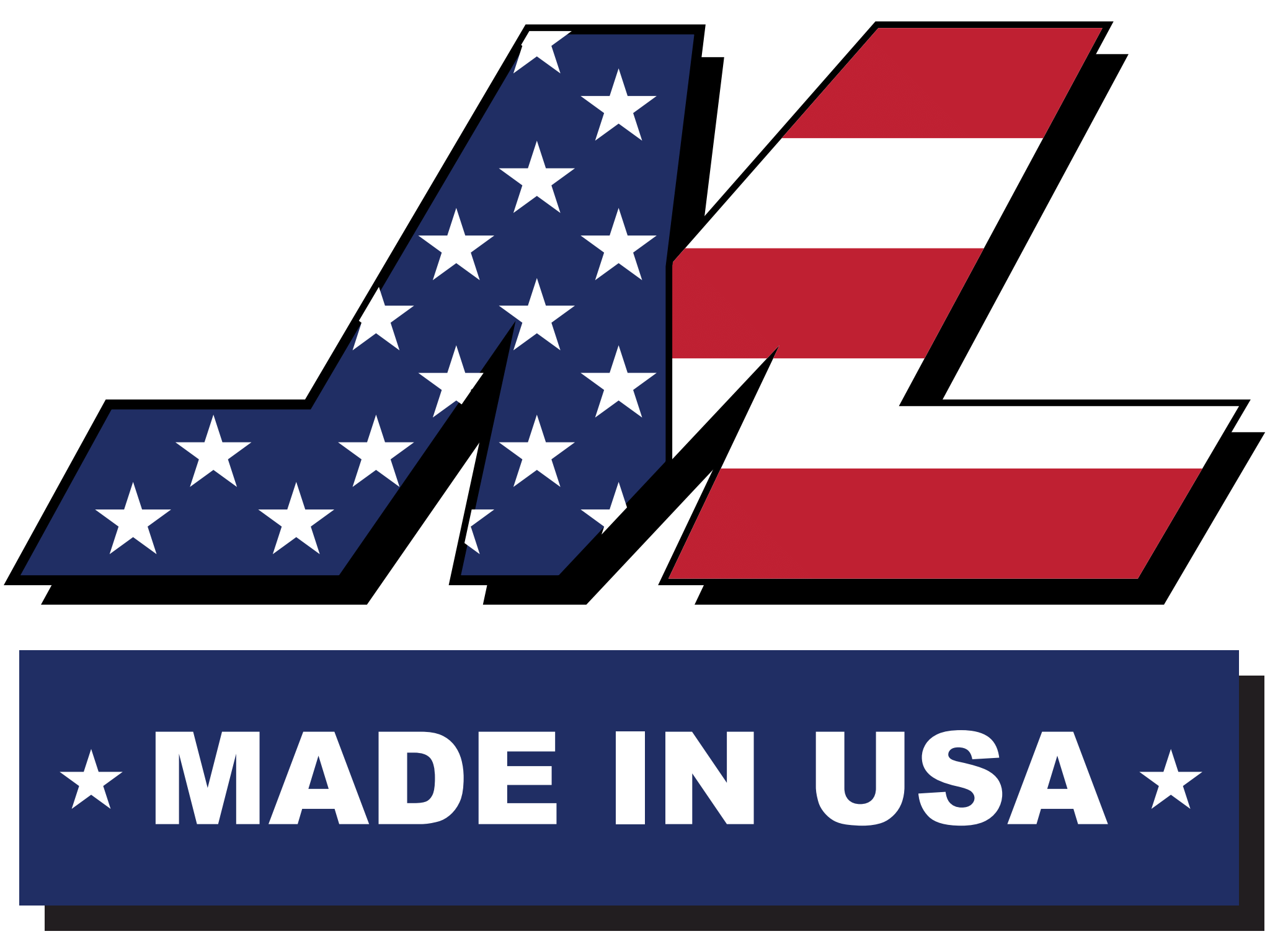### P-Style Coupler

P style coupler bodies are made of heavy duty steel and have 8 locking balls for better retention of plugs. P style couplers come with a 3/8" basic flow size, maximum of 300 PSI and air flow of 68 SCFM. Complete with a Buna-N seal.

P style coupler bodies are made of heavy duty steel and have 8 locking balls for better retention of plugs. P style couplers come with a 3/8" basic flow size, maximum of 300 PSI and air flow of 68 SCFM. Complete with a Buna-N seal.

 Type of Hose : Type of fiiting : Thread Size : SCFM : Air Flow 68 SCFM PSI range :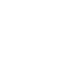### FREE SHIPPING

When you spend \$75+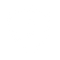### WARRANTY INFO

Offering a range of policies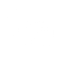### NEED HELP

Contact our support team today### FIND A DISTRIBUTOR

Locate the closest inventory